# 3.2. Inkplate Arduino¶

To get started with Inkplate in Arduino IDE first select right board and include Inkplate.h
```#include "Inkplate.h"
```

## 3.2.1. System Functions¶

### 3.2.1.1. Inkplate object initialization¶

To use any of Inkplates functionalities in Arduino IDE you need to include Inkplate header, as mentioned on the Index and Get Started pages. After you’ve done that you can create a new instance of the Inkplate object, like this:

```Inkplate display(INKPLATE_1BIT);
```

or

```Inkplate display(INKPLATE_3BIT);
```

depending on what you need, monochrome (INKPLATE_1BIT) of grayscale (INKPLATE_3BIT) functionality.

In here given examples, Inkplate object will always be named display, if not said otherwise. After calling this below your “#include” lines, you have access to all Inkplate functionality as display object methods.

### 3.2.1.2. begin() method¶

Before calling any display method you must call .begin() like this:
```display.begin();
```
• Description:

If you forget to do this most method calls will result in core panick and esp32 resetting. For most use cases this function is called in Arduino’s setup function. After you’ve done this you can proceed calling all other methods described below.

### 3.2.1.3. Inkplate::sdCardInit();¶

• Method prototype (as seen in System.h):
```int sdCardInit();
```
• Arguments and return value:
No Arguments

Returns 0 if card initialization unsuccessful, else some number which casts to true.

• Description:
Used to initialize SD card interface.
Must be called before using SD card functionality like SdFile::read();

### 3.2.1.4. Inkplate::getSdFat();¶

• Method prototype (as seen in System.h):
```SdFat Inkplate::getSdFat()
```
• Arguments and return value:
No Arguments

Returns SdFat object.

• Description:
See SdFat library documentation for use examples.

### 3.2.1.5. Inkplate::getSPI();¶

• Method prototype (as seen in System.h):
```SPIClass getSPI();
```
• Arguments and return value:
No Arguments

Returns SPIClass object.

### 3.2.1.6. Inkplate::getPanelState();¶

• Method prototype (as seen in System.h):
```uint8_t getPanelState();
```
• Arguments and return value:
No Arguments.

Returns 1 if eink panel is on, and 0 if it’s off.

• Description:
Used to see if the panel is on.
• Example:
```Serial.print(display.getPanelState(), DEC);
```

• Method prototype (as seen in System.h):
```uint8_t readTouchpad(uint8_t);
```
• Arguments and return value:

Returns state of the desired pad.

• Description:
• Example:
```if (display.readTouchpad(PAD1))
{
//Do something
}
```

• Method prototype (as seen in System.h):
```int8_t readTemperature();
```
• Arguments and return value:
No arguments.

Returns panel temperature at the last refresh.

• Description:
Can be used to determine temperature roughly.
Keep in mind that the returned value was measured at the time of the last screen refresh.
• Example:
```Serial.print(display.readTemperature(), DEC);
```

• Method prototype (as seen in System.h):
```double readBattery();
```
• Arguments and return value:
No Arguments.

Returns battery voltage as a double.

• Description:
Function used to determine battery voltage.
Can be used to display how much more time will the device be on.
• Example:
```double voltage = display.readBattery();
```

### 3.2.1.10. Inkplate::einkOff();¶

• Method prototype (as seen in Inkplate.h):
```void einkOff(void);
```
• Arguments and return value:
No Arguments.

Returns nothing.

• Description:
Turns the panel off to save energy.
• Example:
```display.einkOff();
```

### 3.2.1.11. Inkplate::einkOn();¶

• Method prototype (as seen in Inkplate.h):
```void einkOn(void);
```
• Arguments and return value:
No Arguments.

Returns nothing.

• Description:
Turns the panel back on.
• Example:
```display.einkOn();
```

## 3.2.2. Drawing Functions¶

### 3.2.2.1. Inkplate::drawPixel();¶

• Method prototype (as seen in Graphics.h):
```void drawPixel(int16_t x0, int16_t y0, uint16_t color);
```
• Arguments and return value:
int16_t x0 - x coordinate of pixel, [0, 799] in rotations 2, 4 and [0, 599] in 1, 3
int16_t y0 - y coordinate of pixel, [0, 599] in rotations 2, 4 and [0, 799] in 1, 3
uint16_t color - pixel color, in 3 bit mode in range [0, 7]

Returns nothing.

• Description:
Most basic drawing command in the library is .drawPixel();
Draws one pixel at x0, y0 in desired color.
Requires Inkplate::display() to be called afterwards to update the screen,
See below.
• Example:
```display.drawPixel(100, 50, BLACK);
```
• Result:
Here is what the code above produces:
Quite small, isn’t it.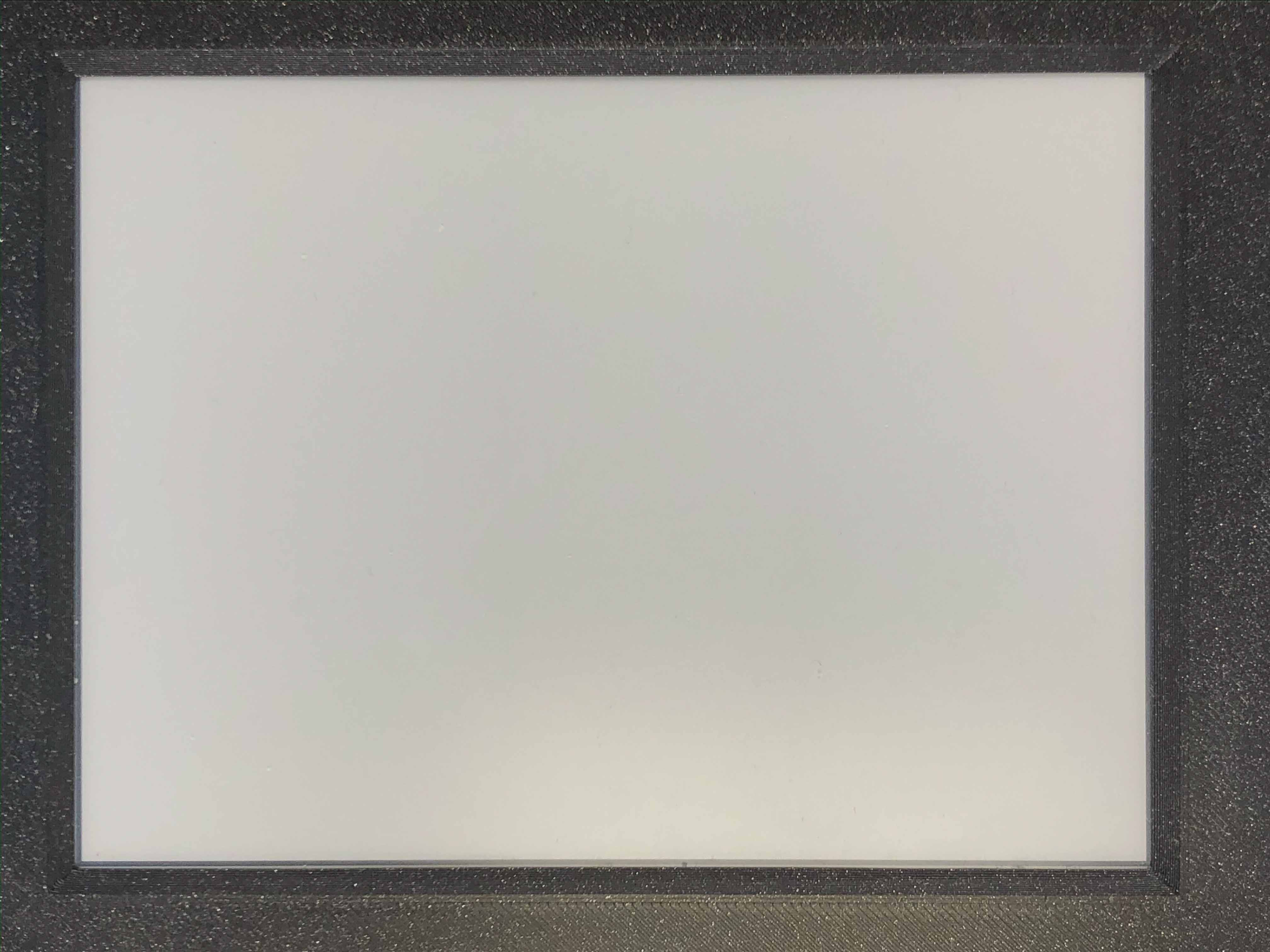### 3.2.2.2. Inkplate::display();¶

• Method prototype (as seen in Inkplate.h):
```void display();
```
• Arguments and return value:
No Arguments

Returns nothing.

• Description:
Displays all data in frame buffer to screen.
• Example:
```//Any drawing code
display.drawPixel(10, 100, BLACK);

display.display();
```

### 3.2.2.3. Inkplate::clearDisplay();¶

• Method prototype (as seen in Inkplate.h):
```void clearDisplay();
```
• Arguments and return value:
No Arguments

Returns nothing.

• Description:
Clears all data in buffer. Call display() after this to update/clear display.
• Example:
```display.clearDisplay();
display.display();
```

### 3.2.2.4. Inkplate::partialUpdate();¶

• Method prototype (as seen in Inkplate.h):
```void partialUpdate();
```
• Arguments and return value:
No Arguments

Returns nothing.

• Description:
Updates only the changed parts of the screen. (monochrome/INKPLATE_1BIT mode only!)
After a few updates creates blurry parts of the screen.
Fixed by calling Inkplate::clean();
• Example:
```display.drawPixel(100, 50, BLACK);

display.partialUpdate();

display.drawPixel(100, 100, BLACK);
```

### 3.2.2.5. Inkplate::setRotation();¶

• Method prototype (as seen in Graphics.h):
```void setRotation(uint8_t r);
```
• Arguments and return value:
uint8_t r - screen rotation.

Returns nothing.

• Description:
Rotates the screen to be used in different orientations.
Default is 2, to flip 180 input 4
1 and 3 are for portait mode.
Once flipped coordinate space remains to have the origin in the top left corner.
• Example:
```display.setRotation(3);

display.setCursor(100, 100);
display.print("INKPLATE");
```
• Result:
Here is what the code above produces: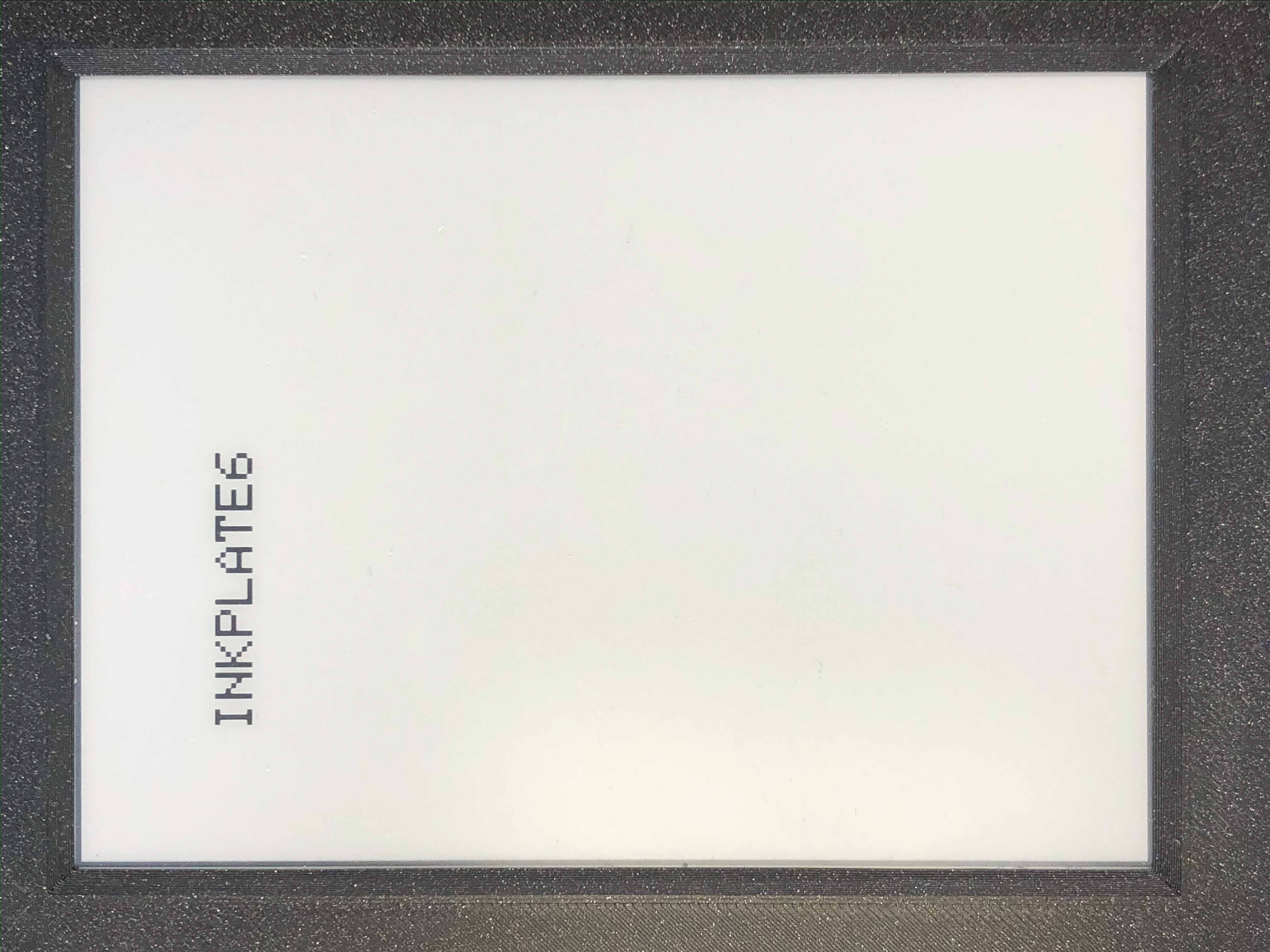### 3.2.2.6. Inkplate::selectDisplayMode();¶

• Method prototype (as seen in Graphics.h):
```void selectDisplayMode(uint8_t _mode)
```
• Arguments and return value:
uint8_t _mode - New display mode, INKPLATE_1BIT or INKPLATE_3BIT.

Returns nothing.

• Description:
Changes the screen mode to from monochrome to 3 bit grayscale or vice versa.
• Example:
```display.selectDisplayMode(INKPLATE_3BIT);
```

### 3.2.2.7. Inkplate::getDisplayMode();¶

• Method prototype (as seen in Graphics.h):
```uint8_t getDisplayMode();
```
• Arguments and return value:
No arguments.

Returns currently set display mode.

• Description:
Used to determine which display mode is currently used.
Returns INKPLATE_1BIT or INKPLATE_3BIT.
• Example:
```if(display.getDisplayMode() == INKPLATE_3BIT)
Serial.println("I'm in grayscale mode!");
```

### 3.2.2.8. Inkplate::drawImage();¶

• Method prototype (as seen in Image.h):
```bool drawImage(const char *path, int x, int y, bool dither = 1, bool invert = 0);
bool drawImage(const String path, int x, int y, bool dither = 1, bool invert = 0);
bool drawImage(const uint8_t *buf, int x, int y, int16_t w, int16_t h, uint8_t c = BLACK, uint8_t bg = 0xFF);
bool drawImage(const char *path, const Format &format, const int x, const int y, const bool dither = 1, const bool invert = 0);
bool drawImage(const String path, const Format &format, const int x, const int y, const bool dither = 1, const bool invert = 0);
bool drawImage(const char *path, const Format &format, const Position &position, const bool dither = 1, const bool invert = 0);
```
• Arguments and return value:
const char *path - Path to file.
int x - x coordinate to draw the image at
int y - y coordinate to draw the image at
bool dither - to dither the image or not
bool invert - invert all colors, defaults to false

const String path - Path to file.
int x - x coordinate to draw the image at
int y - y coordinate to draw the image at
bool dither - to dither the image or not
bool invert - invert all colors, defaults to false

const uint8_t *p - Buffer to draw from.
int x - x coordinate to draw the image at
int y - y coordinate to draw the image at
int16_t w - x coordinate to draw the image at
int16_t h - y coordinate to draw the image at
bool dither - to dither the image or not
bool invert - invert all colors, defaults to false
uint8_t c - color to draw 1 pixels if in BW mode
uint8_t bg - color to draw all 0 pixels if in BW mode.
const char *path - Path to file.
const Format &format - image format (bmp, jpeg, png).
int x - x coordinate to draw the image at
int y - y coordinate to draw the image at
bool dither - to dither the image or not
bool invert - invert all colors, defaults to false
const String *path - Path to file.
const Format &format - image format (bmp, jpeg, png).
int x - x coordinate to draw the image at
int y - y coordinate to draw the image at
bool dither - to dither the image or not
bool invert - invert all colors, defaults to false
const char *path - Path to file.
const Format &format - image format (bmp, jpeg, png).
const Position &position - image position (Center, TopLeft, BottomLeft, TopRight, BottomRight, _npos)
bool dither - to dither the image or not
bool invert - invert all colors, defaults to false

Returns 0 if error occured, else returns 1.

• Description:
Should always have Inkplate::sdCardInit() called before if file is from SD.
Can draw all kinds of images, but they should have a file extensions in them.
Can draw from web if path starts with http:// or https:// or if not from SD.
Draws bmp, png and jpeg images.
Automatically adjusts for current display mode.

### 3.2.2.9. Inkplate::drawBitmapFromSD();¶

• Method prototype (as seen in Image.h):
```[[deprecated("Use drawImage, as this will soon become a private method.")]]
int drawBitmapFromSD(SdFile *p, int x, int y, bool dither = false, bool invert = false);
int drawBitmapFromSD(char *fileName, int x, int y, bool dither = false, bool invert = false);
```
• Arguments and return value:
SdFile *p - SdFile pointer to draw to screen
int x - x coordinate to draw the image at
int y - y coordinate to draw the image at
bool dither - to dither the image or not
bool invert - invert all colors, defaults to false

char *fileName - filename of the bmp on the sd card
int x - x coordinate to draw the image at
int y - y coordinate to draw the image at
bool dither - to dither the image or not
bool invert - invert all colors, defaults to false

Returns 0 if error occured, else returns 1.

• Description:
Should always have Inkplate::sdCardInit() called before.
Draws a bitmap image from sd card to screen.
Image can currently have 1, 4, 8 or 24 bit color depth.
24 or 8 bit ones can be dithered, else the argument is ignored.
Info on dithering: dithering
• Example:
```if (display.sdCardInit())
{
display.partialUpdate();
if(!display.drawBitmapFromSD("pandaImage.bmp", 0, 0)) {
display.println("Image open error");
display.display();
}
}
```
• Result:
Here is what the code above produces: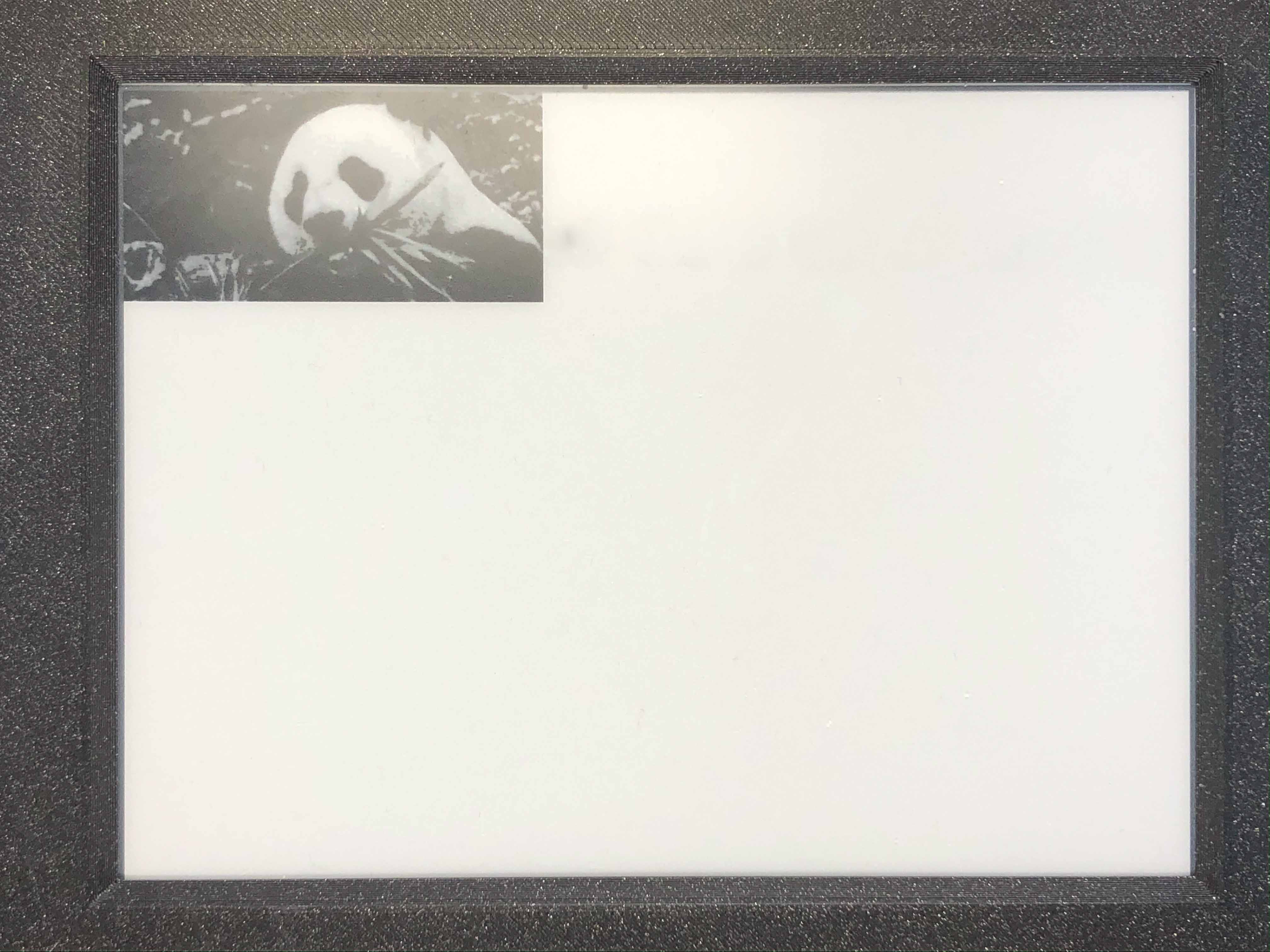### 3.2.2.10. Inkplate::drawBitmapFromWeb();¶

• Method prototype (as seen in Image.h):
```[[deprecated("Use drawImage, as this will soon become a private method.")]]
int drawBitmapFromWeb(WiFiClient *s, int x, int y, int len, bool dither = false, bool invert = false);
int drawBitmapFromWeb(char *url, int x, int y, bool dither = false, bool invert = false);
```
• Arguments and return value:
WiFiClient *s - WiFiClient stream to dowload image from.
int x - x coordinate at which to display the image.
int y - y coordinate at which to display the image.
int len - file size (header included).
bool dither - flag indicating to dither the image
bool invert - invert all image colors.

char *url - url of the image.
int x - x coordinate at which to display the image.
int y - y coordinate at which to display the image.
bool dither - flag indicating to dither the image
bool invert - invert all image colors.

Returns 0 if failed and 1 if successful.

• Description:
Draws an image from the web.
• Example:
```if(!display.drawBitmapFromWeb("https://varipass.org/neowise_mono.bmp", 0, 0, true)) {
display.println("Image open error");
display.display();
}
```
• Result:
Here is what the code above produces: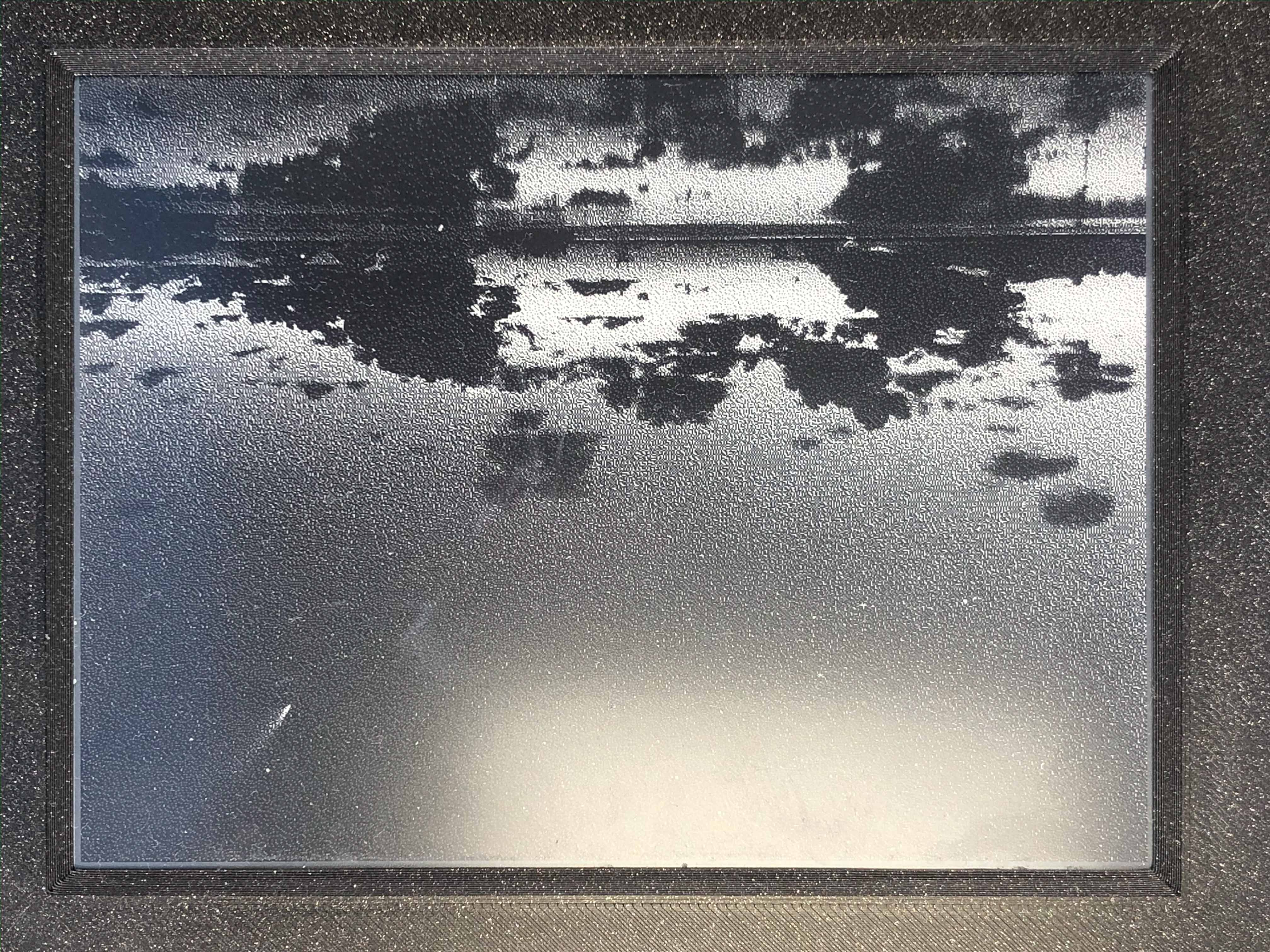### 3.2.2.11. Inkplate::drawThickLine();¶

• Method prototype (as seen in Shapes.h):
```void drawThickLine(int x1, int y1, int x2, int y2, int color, float thickness);
```
• Arguments and return value:
int x1 - x coordinate of line start, [0, 799] in rotations 2, 4 and [0, 599] in 1, 3
int y1 - y coordinate of line start, [0, 599] in rotations 2, 4 and [0, 799] in 1, 3
int x2 - x coordinate of line end, [0, 799] in rotations 2, 4 and [0, 599] in 1, 3
int y2 - y coordinate of line end, [0, 599] in rotations 2, 4 and [0, 799] in 1, 3
int color - line color, in 3 bit mode in range [0, 7]
float thickness - line thickness in pixels

Returns nothing.

• Description:
For drawing thick lines.
• Example:
```display.drawThickLine(random(0, 799), random(0, 599), random(0, 799), random(0, 599), BLACK, (float)random(1, 20));
```
• Result:
Here is what the code above produces: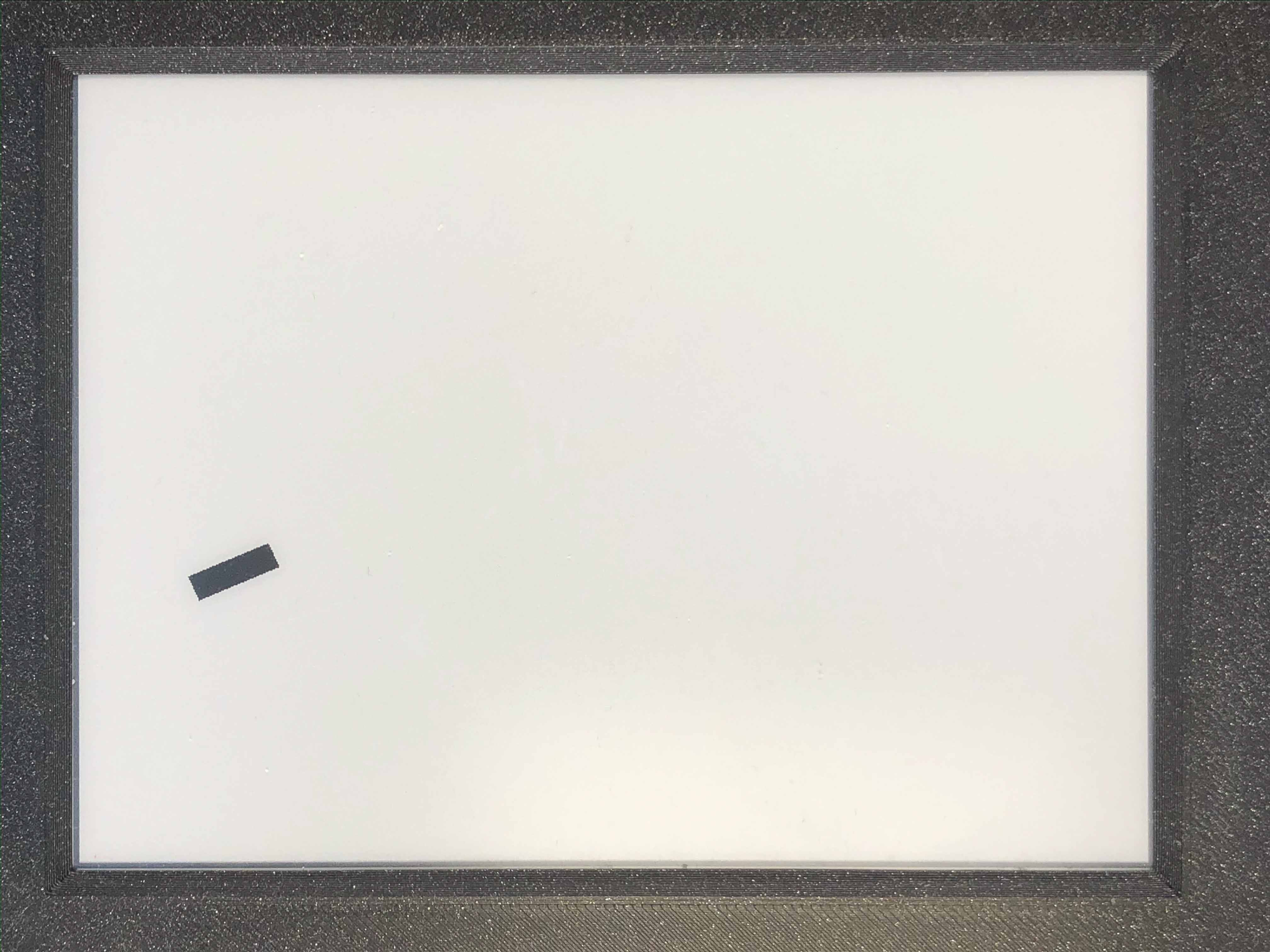• Method prototype (as seen in Shapes.h):
```void drawGradientLine(int x1, int y1, int x2, int y2, int color1, int color2, float thickness = -1);
```
• Arguments and return value:
int x1 - x coordinate of line start, [0, 799] in rotations 2, 4 and [0, 599] in 1, 3
int y1 - y coordinate of line start, [0, 599] in rotations 2, 4 and [0, 799] in 1, 3
int x2 - x coordinate of line end, [0, 799] in rotations 2, 4 and [0, 599] in 1, 3
int y2 - y coordinate of line end, [0, 599] in rotations 2, 4 and [0, 799] in 1, 3
int color1 - start line color, in 3 bit mode in range [0, 7]
int color2 - start line color, in 3 bit mode in range [0, 7]
float thickness - line thickness, defaults to -1 meaning use normal, non thick, line.

Returns nothing.

• Description:
color1 should always be less than color2.
• Example:
```int startColor = random(0, 7);
int endColor = random(startColor, 7);
display.drawGradientLine(random(0, 799), random(0, 599), random(0, 799), random(0, 599), startColor, endColor, (float)random(1, 20));
```
• Result:
Here is what the code above produces: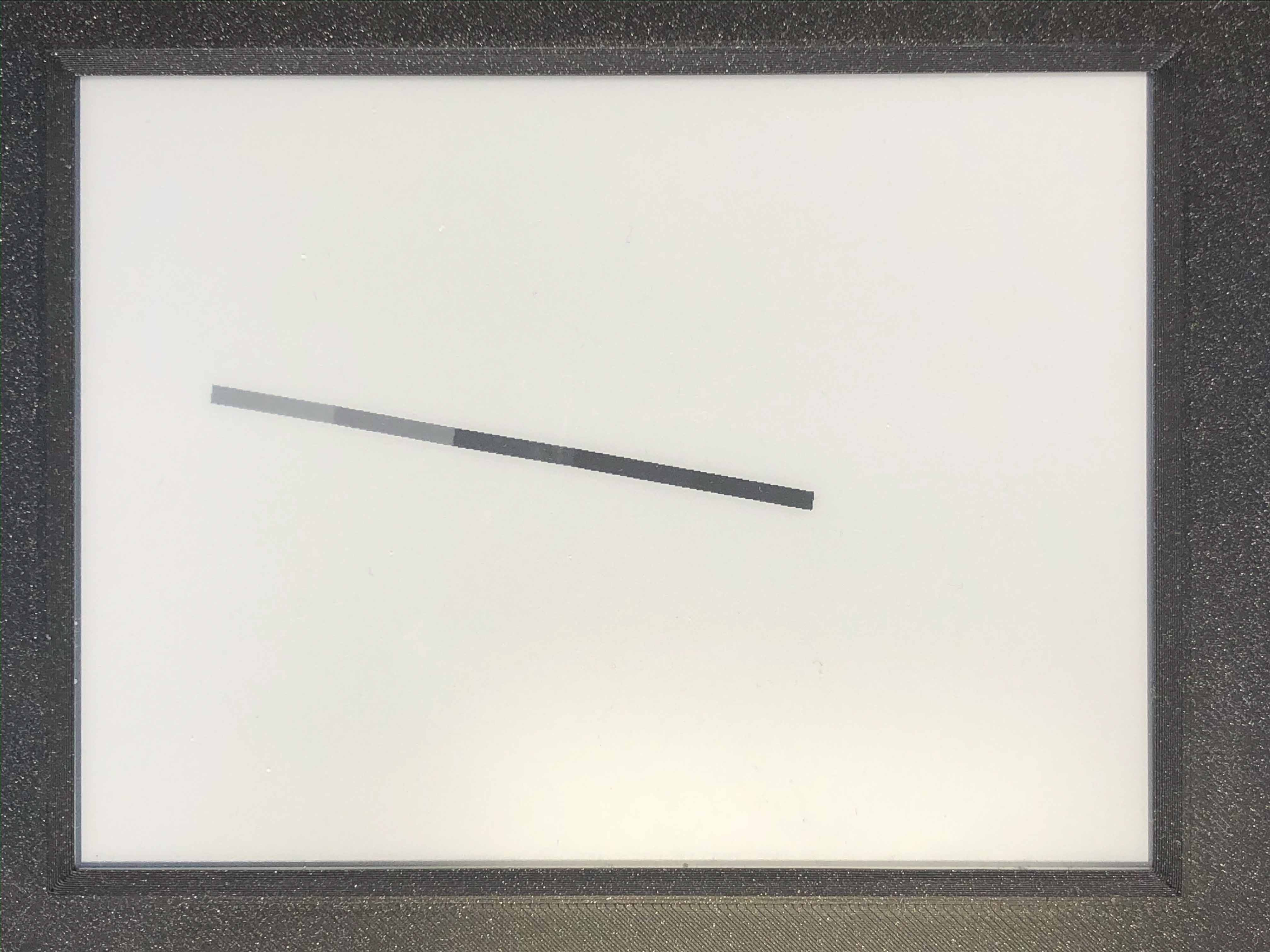### 3.2.2.13. Inkplate::clean();¶

• Method prototype (as seen in Inkplate.h):
```void clean();
```
• Arguments and return value:
No arguments.

Returns nothing.

• Description:
Cleans the actual screen of any possible burn in.
Should not be used in intervals less than 5 seconds.
• Example:
```display.clean();
```

### 3.2.2.14. Inkplate::drawFastVLine();¶

• Method prototype (as seen in Graphics.h):
```void drawFastVLine(int16_t x, int16_t y, int16_t h, uint16_t color);
```
• Arguments and return value:
int16_t x - x coordinate of the line start point
int16_t y - y coordinate of the line start point
int16_t h - line height
uint16_t color - line color

Returns nothing.

• Description:
Draw a perfectly vertical line.
Garantees to be faster than regular line draw.
• Example:
```display.drawFastVLine(100, 100, 400, 0);
```
• Result:
Here is what the code above produces: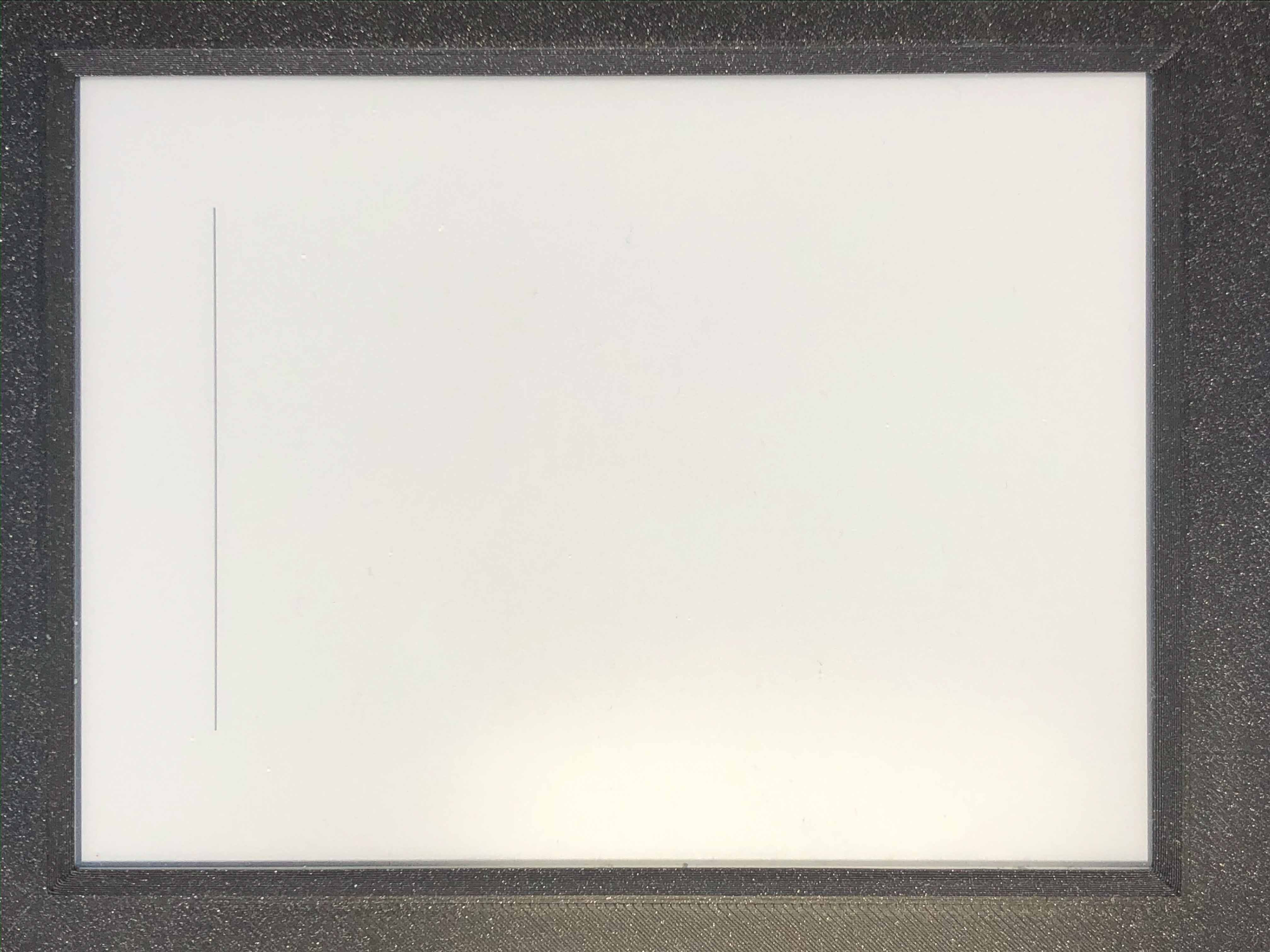### 3.2.2.15. Inkplate::drawFastHLine();¶

• Method prototype (as seen in Graphics.h):
```void drawFastHLine(int16_t x, int16_t y, int16_t w, uint16_t color);
```
• Arguments and return value:
int16_t x - x coordinate of the line start point
int16_t y - y coordinate of the line start point
int16_t w - line width
uint16_t color - line color

Returns nothing.

• Description:
Draw a perfectly horizontal line.
Garantees to be faster than regular line draw.
• Example:
```display.drawFastHLine(100, 100, 600, 0);
```
• Result:
Here is what the code above produces: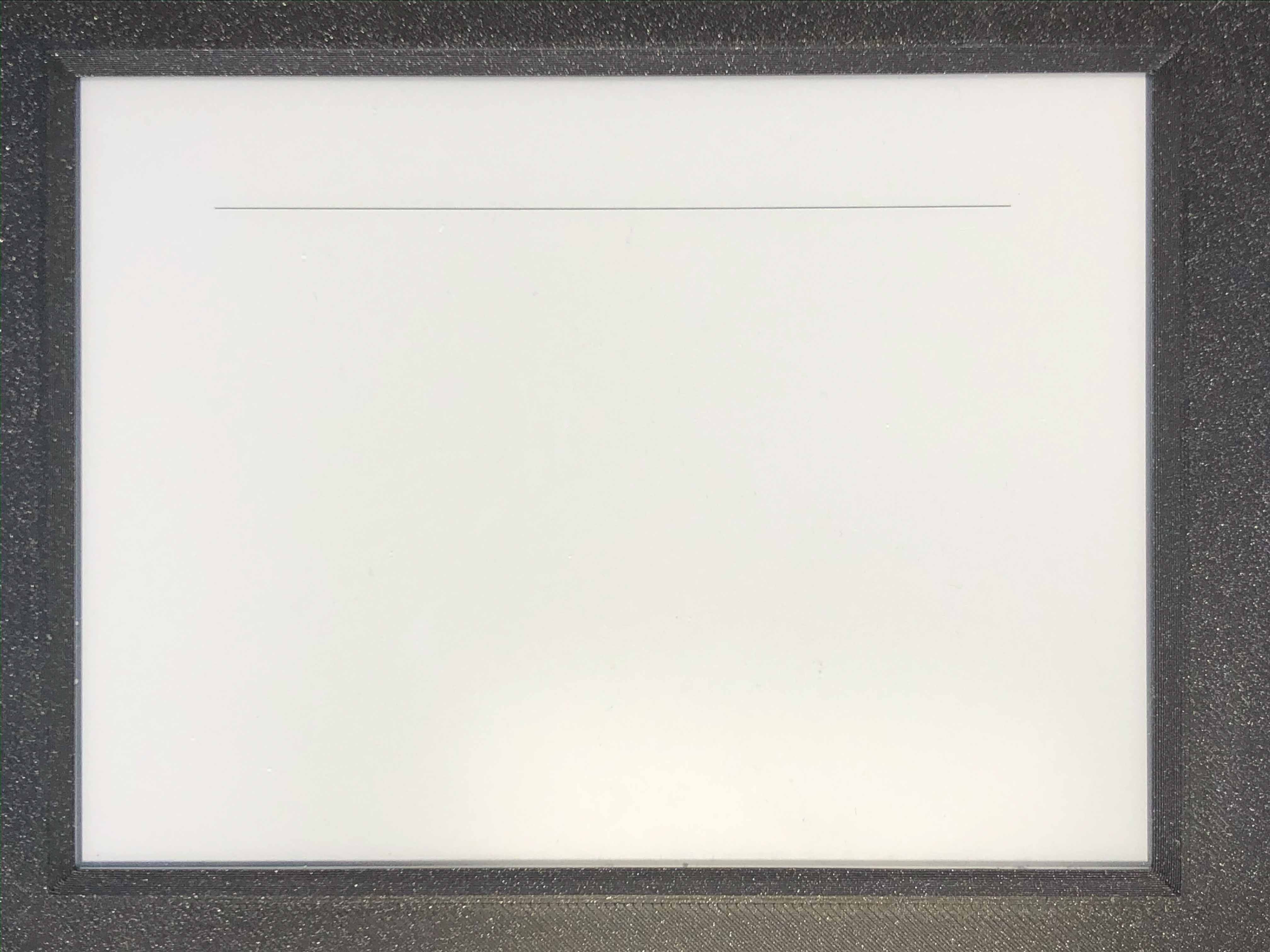### 3.2.2.16. Inkplate::fillRect();¶

• Method prototype (as seen in Graphics.h):
```void fillRect(int16_t x, int16_t y, int16_t w, int16_t h, uint16_t color);
```
• Arguments and return value:
int16_t x - x coordinate of the rectangle
int16_t y - y coordinate of the rectangle
int16_t w - rectangle width
int16_t h - rectangle height
uint16_t color - rectanle color, in range [0, 6]

Returns nothing.

• Description:
Draws a filled rectangle on the screen.
• Example:
```display.fillRect(random(0, 799), random(0, 599), 30, 30, random(0, 7));
```
• Result:
Here is what the code above produces: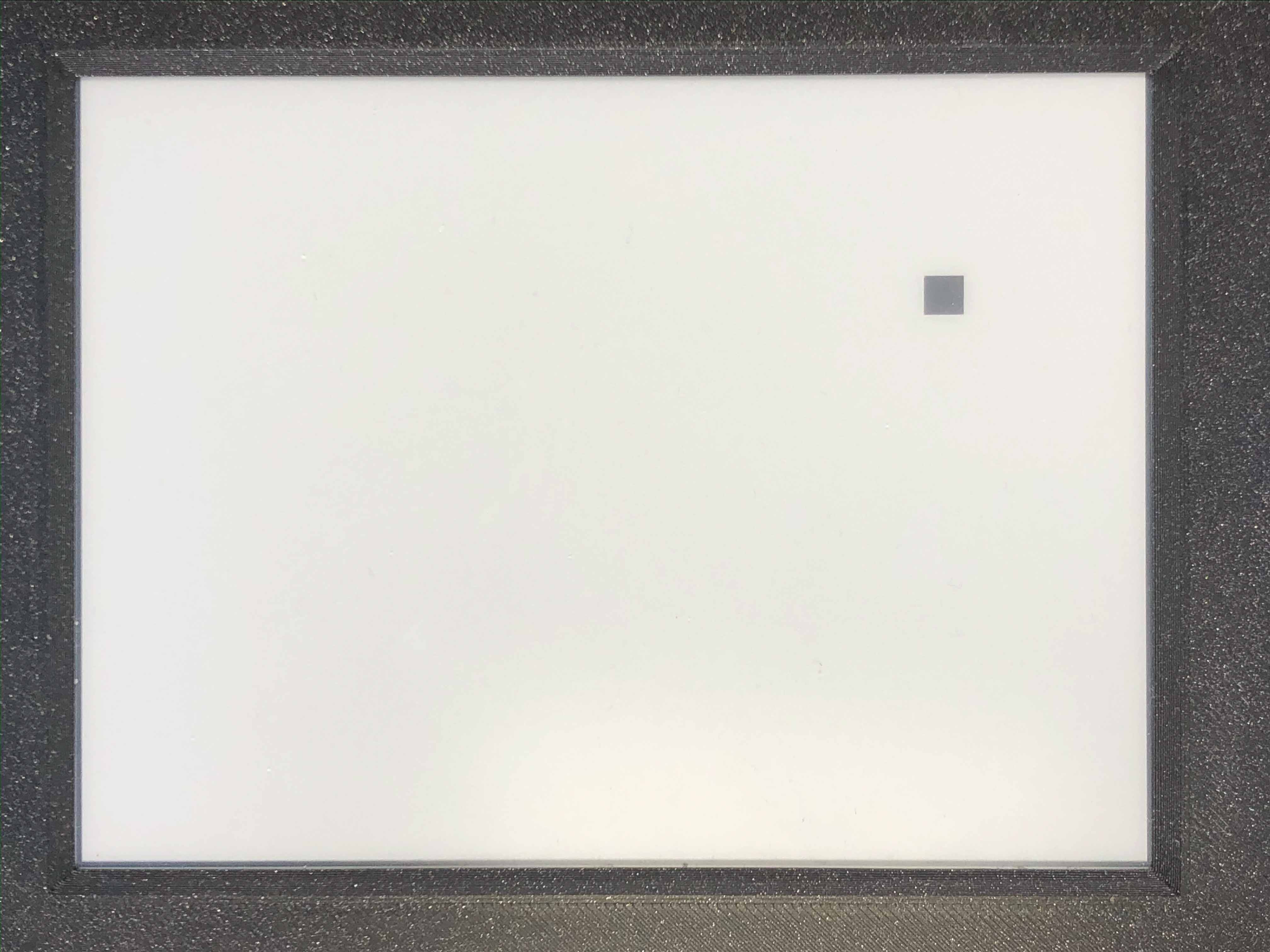### 3.2.2.17. Inkplate::fillScreen();¶

• Method prototype (as seen in Adafruit_GFX.h):
```void fillScreen(uint16_t color);
```
• Arguments and return value:
uint16_t color - color of the screen after filling.

Returns nothing.

• Description:
Fills the whole screen to a solid color.
• Example:
```display.fillScreen(0);
```
• Result:
Here is what the code above produces: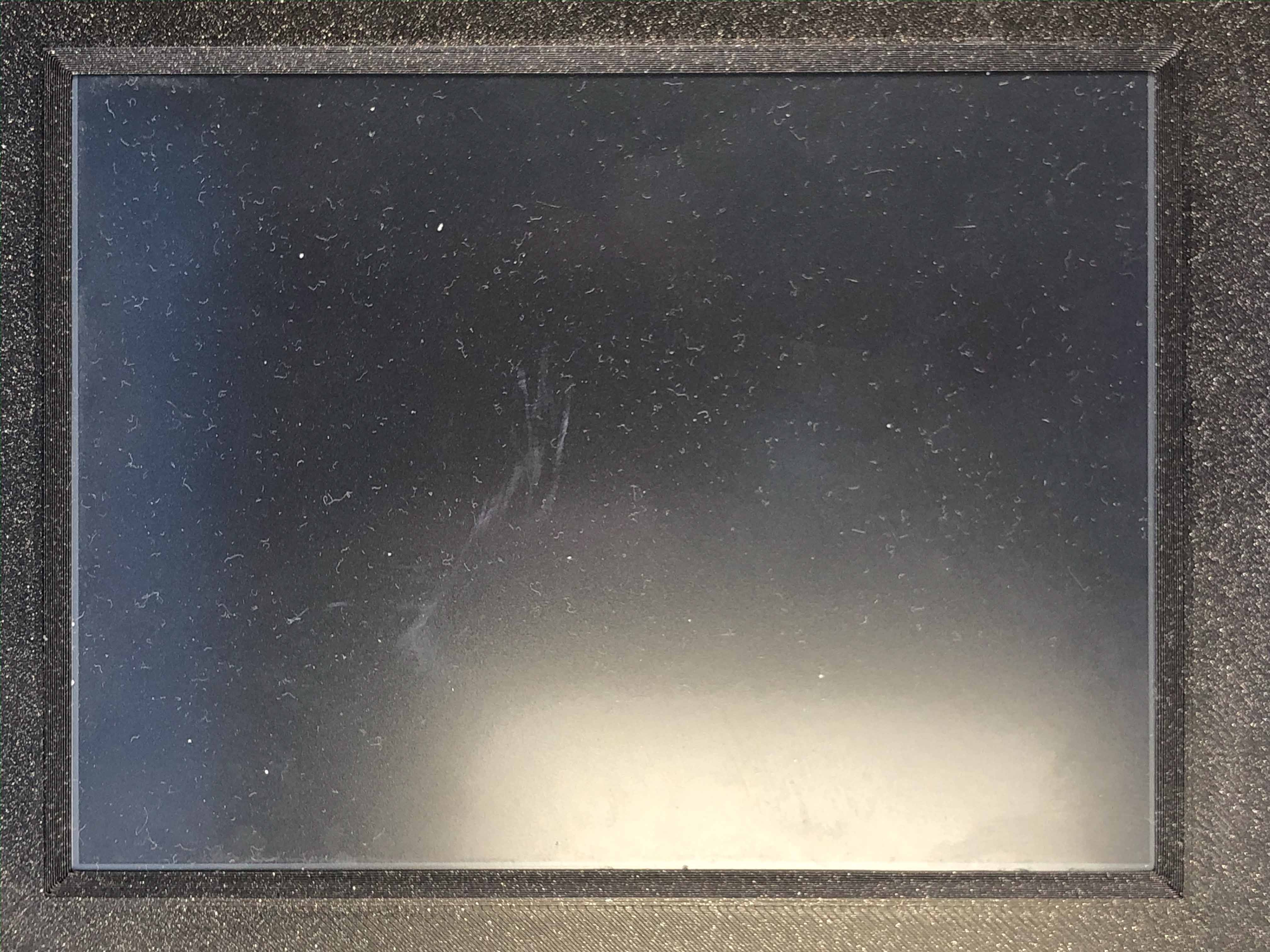### 3.2.2.18. Inkplate::drawLine();¶

• Method prototype (as seen in Adafruit_GFX.h):
```void drawLine(int16_t x0, int16_t y0, int16_t x1, int16_t y1, uint16_t color);
```
• Arguments and return value:
int16_t x0 - Start point x coordinate.
int16_t y0 - Start point y coordinate.
int16_t x1 - End point x coordinate.
int16_t y1 - End point y coordinate.
uint16_t color - Line color.

Returns nothing.

• Description:
General purpose line drawing function.
If the line is vertical or horizontal it is recommended to use drawFastHLine or drawFastVLine,
although drawLine automatically checks and uses faster drawing function if needed.
• Example:
```//Diagonal lines
display.drawLine(0, 0, 799, 599, 0);
display.drawLine(799, 0, 0, 599, 0);
```
• Result:
Here is what the code above produces: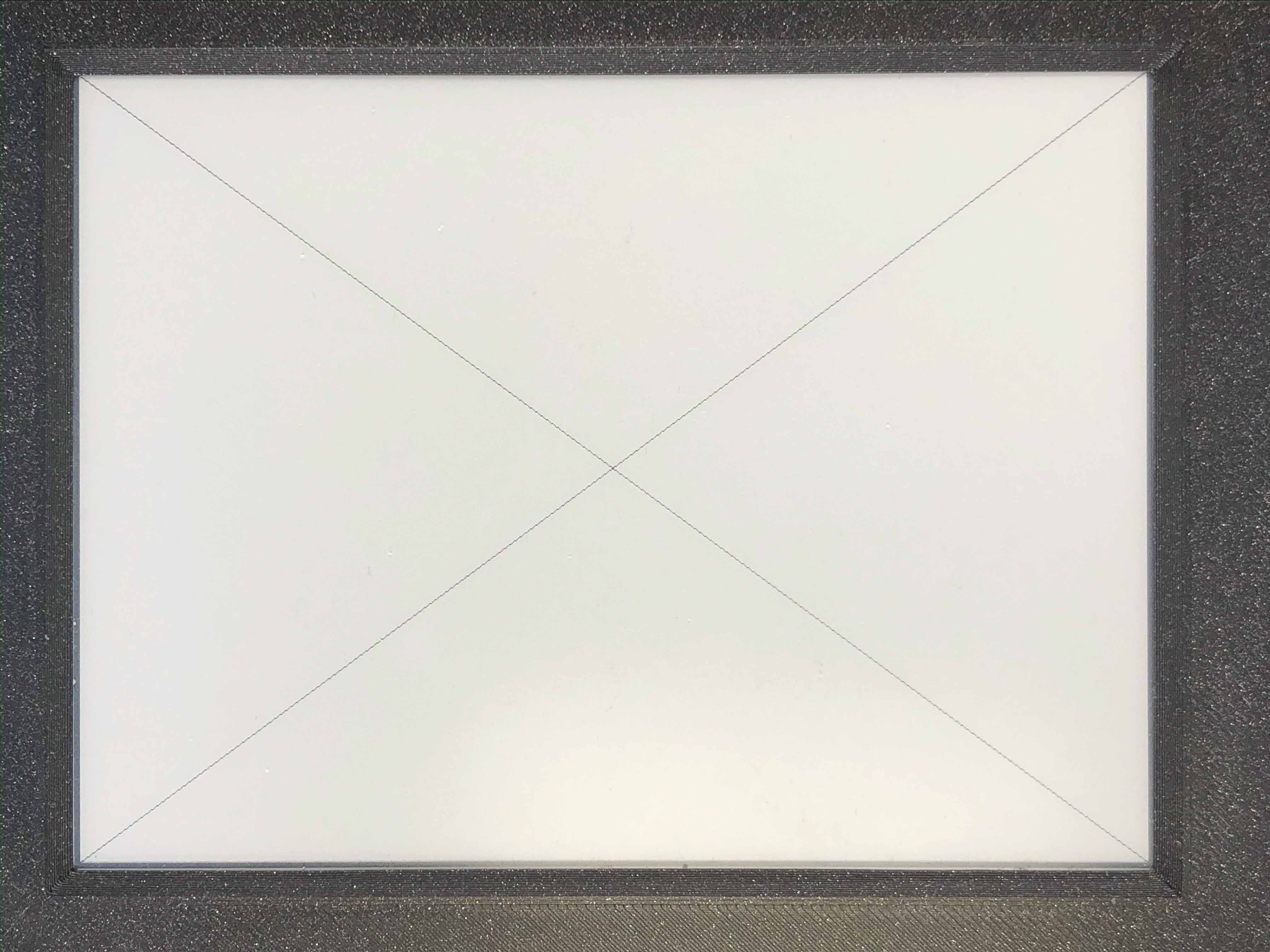### 3.2.2.19. Inkplate::drawRect();¶

• Method prototype (as seen in Adafruit_GFX.h):
```void drawRect(int16_t x, int16_t y, int16_t w, int16_t h, uint16_t color);
```
• Arguments and return value:
int16_t x - Rectangle x coordinate.
int16_t y - Rectangle y coordinate.
int16_t w - Rectangle width.
int16_t h - Rectangle height.
uint16_t color - Rectangle color (edges only, see fillRect for fully filled one).

Returns nothing.

• Description:
Draws and empty (not filled) rectangle.
• Example:
```display.drawRect(200, 200, 400, 300, 0);
```
• Result:
Here is what the code above produces: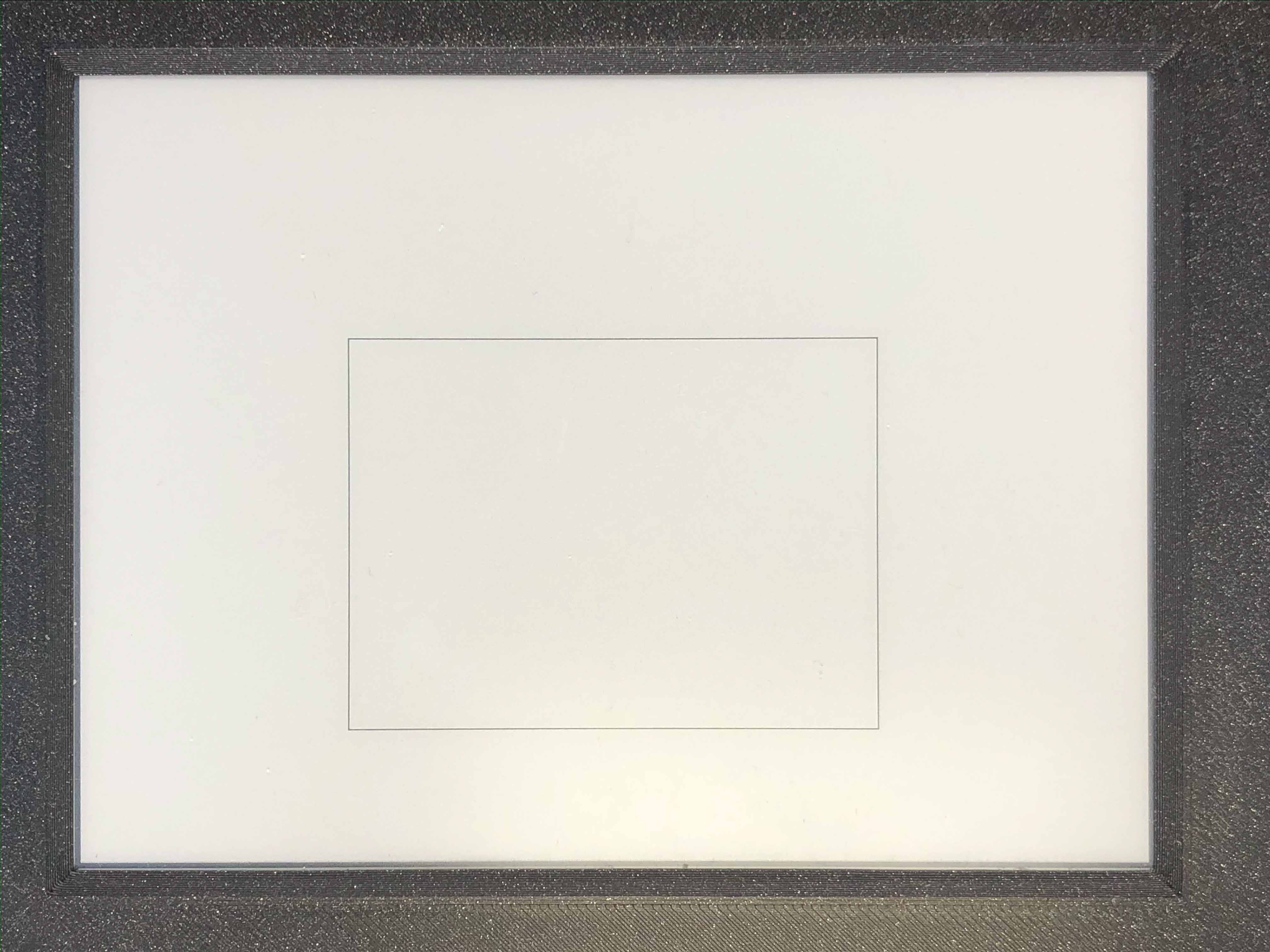### 3.2.2.20. Inkplate::drawElipse();¶

• Method prototype (as seen in Shapes.h):
```void drawElipse(int rx, int ry, int xc, int yc, int c);
```
• Arguments and return value:
int rx - Elipse X radius.
int ry - Elipse Y radius.
int xc - Elipse center x.
int yc - Elipse center y.
int color - Elipse color (just the edge, see fillElipse for fully filled).

Returns nothing.

• Description:
Draws an empty(not filled) elipse.
• Example:
```display.drawElipse(100, 200, 400, 300, 0);
```

### 3.2.2.21. Inkplate::fillElipse();¶

• Method prototype (as seen in Shapes.h):
```void fillElipse(int rx, int ry, int xc, int yc, int c);
```
• Arguments and return value:
int rx - Elipse X radius.
int ry - Elipse Y radius.
int xc - Elipse center x.
int yc - Elipse center y.
int color - Elipse color.

Returns nothing.

• Description:
Draws an filled elipse.
• Example:
```display.fillElipse(100, 200, 400, 300, 0);
```

### 3.2.2.22. Inkplate::drawPolygon();¶

• Method prototype (as seen in Shapes.h):
```void drawPolygon(int *x, int *y, int n, int color);
```
• Arguments and return value:
int *x - Polygon points X coordinates.
int *y - Polygon points Y coordinates.
int n - Number of points.
int color - Elipse color (just the edge, see fillElipse for fully filled).

Returns nothing.

• Description:
Draws an empty(not filled) polygon.
• Example:
```display.drawPolygon(xt, yt, n, 0);
```

### 3.2.2.23. Inkplate::fillPolygon();¶

• Method prototype (as seen in Shapes.h):
```void fillPolygon(int *x, int *y, int n, int color);
```
• Arguments and return value:
int *x - Polygon points X coordinates.
int *y - Polygon points Y coordinates.
int n - Number of points.
int color - Elipse color (just the edge, see fillElipse for fully filled).

Returns nothing.

• Description:
Draws a filled polygon.
Can be quite slow.
• Example:
```display.fillPolygon(xt, yt, n, 0);
```

### 3.2.2.24. Inkplate::drawCircle();¶

• Method prototype (as seen in Adafruit_GFX.h):
```void drawCircle(int16_t x0, int16_t y0, int16_t r, uint16_t color);
```
• Arguments and return value:
int16_t x0 - Circle center x coordinte.
int16_t y0 - Circle center y coordinate.
uint16_t color - Circle color (just the edge, see fillCircle for fully filled).

Returns nothing.

• Description:
Draws an empty(not filled) circle.
• Example:
```display.drawCircle(400, 300, 75, 0);
```
• Result:
Here is what the code above produces: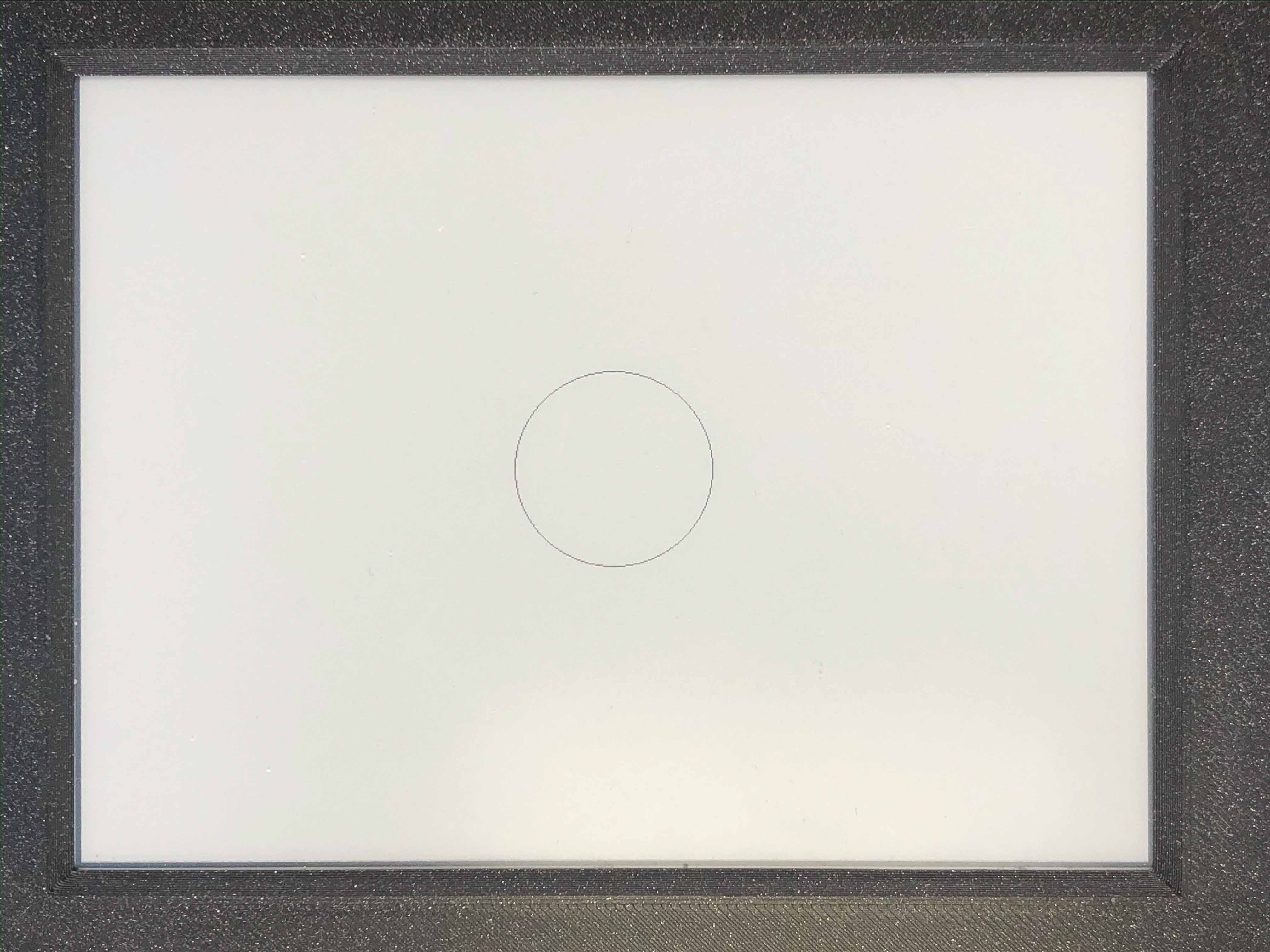### 3.2.2.25. Inkplate::fillCircle();¶

• Method prototype (as seen in Adafruit_GFX.h):
```void fillCircle(int16_t x0, int16_t y0, int16_t r, uint16_t color);
```
• Arguments and return value:
int16_t x0 - Circle center x coordinte.
int16_t y0 - Circle center y coordinate.
uint16_t color - Circle color (fully filled).

Returns nothing.

• Description:
Draws a filled circle to screen in a supplied color.
• Example:
```display.fillCircle(random(0, 799), random(0, 599), 15, random(0, 7));
```
• Result:
Here is what the code above produces: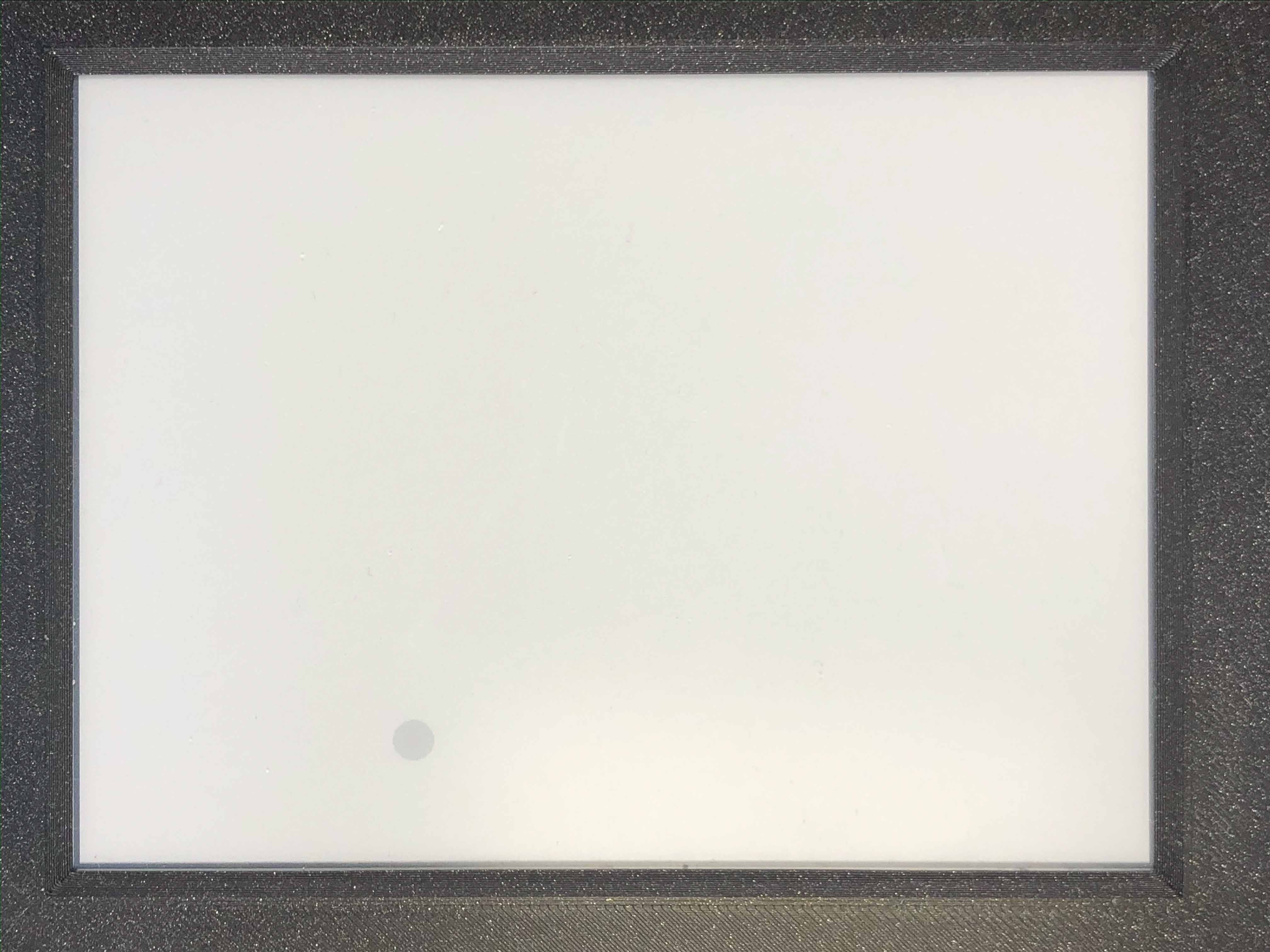### 3.2.2.26. Inkplate::drawTriangle();¶

• Method prototype (as seen in Adafruit_GFX.h):
```void drawTriangle(int16_t x0, int16_t y0, int16_t x1, int16_t y1,
int16_t x2, int16_t y2, uint16_t color);
```
• Arguments and return value:
int16_t x0 - First point x coordinate.
int16_t y0 - First point y coordinate.
int16_t x1 - Second point x coordinate.
int16_t y1 - Second point y coordinate.
int16_t x2 - Third point x coordinate.
int16_t y2 - Third point y coordinate.
uint16_t color - Triangle edge color(see fillTriangle for a fully filled one).

Returns nothing.

• Description:
Draw an empty rectangle to screen.
• Example:
```display.drawTriangle(250, 400, 550, 400, 400, 100, 0);
```
• Result:
Here is what the code above produces: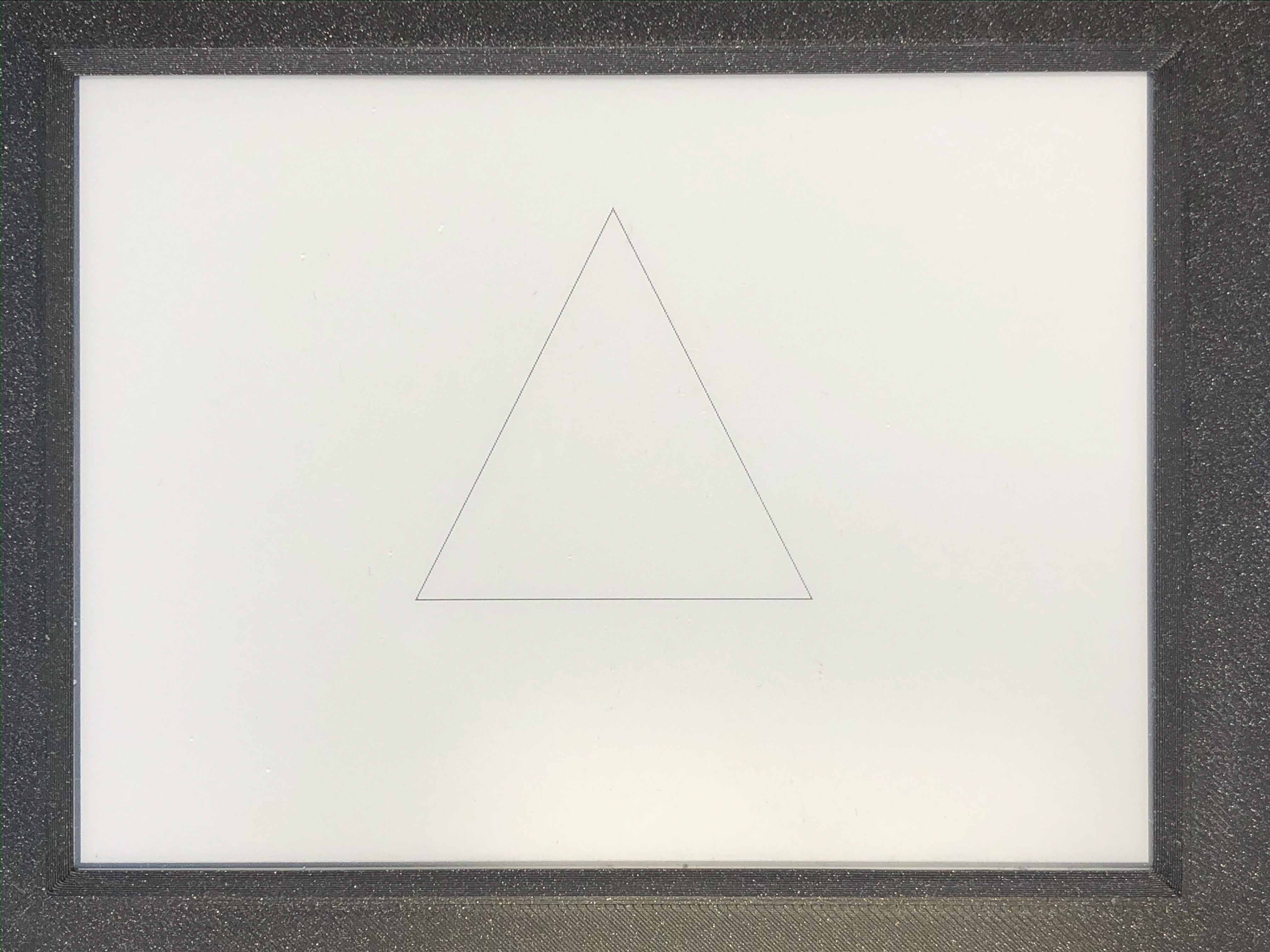### 3.2.2.27. Inkplate::fillTriangle();¶

• Method prototype (as seen in Adafruit_GFX.h):
```void fillTriangle(int16_t x0, int16_t y0, int16_t x1, int16_t y1,
int16_t x2, int16_t y2, uint16_t color);
```
• Arguments and return value:
int16_t x0 - First point x coordinate.
int16_t y0 - First point y coordinate.
int16_t x1 - Second point x coordinate.
int16_t y1 - Second point y coordinate.
int16_t x2 - Third point x coordinate.
int16_t y2 - Third point y coordinate.
uint16_t color - Triangle fill color.

Returns nothing.

• Description:
Draw a rectangle filled with a certain color.
• Example:
```display.fillTriangle(300, 350, 500, 350, 400, 150, 0);
```
• Result:
Here is what the code above produces: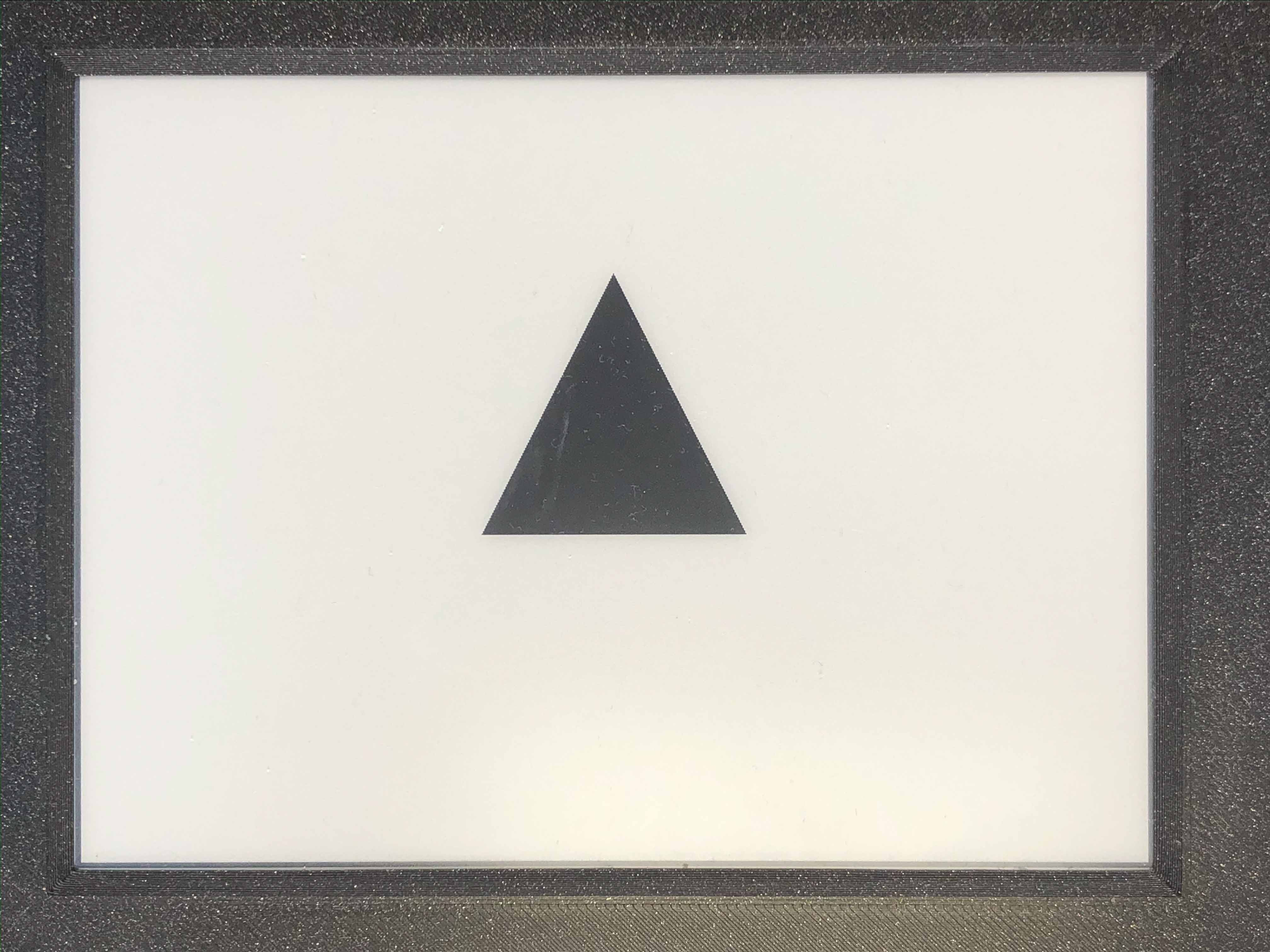### 3.2.2.28. Inkplate::drawRoundRect();¶

• Method prototype (as seen in Adafruit_GFX.h):
```void drawRoundRect(int16_t x0, int16_t y0, int16_t w, int16_t h,
```
• Arguments and return value:
int16_t x0 - Rectangle x coordinate.
int16_t y0 - Rectangle y coordinate.
int16_t w - Rectangle width.
int16_t h - Rectangle height.
uint16_t color - Rectangle edges color (for a fully filled one see fillRoundRect).

Returns nothing.

• Description:
Draws an empty (not filled) rectangle with round edges to screen.
• Example:
```display.drawRoundRect(200, 200, 400, 300, 10, 0);
```
• Result:
Here is what the code above produces: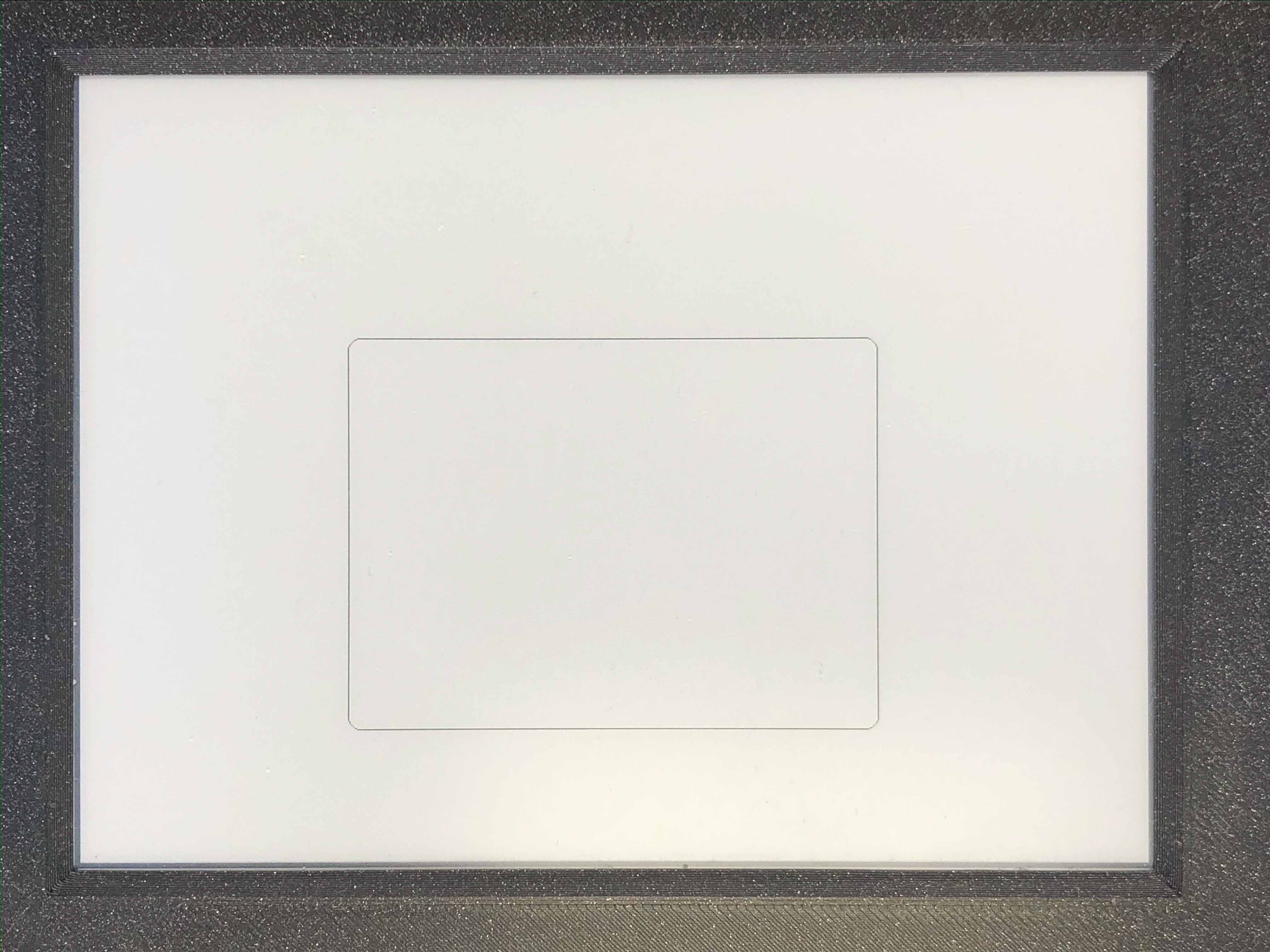### 3.2.2.29. Inkplate::fillRoundRect();¶

• Method prototype (as seen in Adafruit_GFX.h):
```void fillRoundRect(int16_t x0, int16_t y0, int16_t w, int16_t h,
```
• Arguments and return value:
int16_t x0 - Rectangle x coordinate.
int16_t y0 - Rectangle y coordinate.
int16_t w - Rectangle width.
int16_t h - Rectangle height.
uint16_t color - Rectangle fill color.

Returns nothing.

• Description:
Draws a fully filled rectangle with rounded corners to screen.
• Example:
```display.fillRoundRect(200, 200, 400, 300, 10, 0);
```
• Result:
Here is what the code above produces: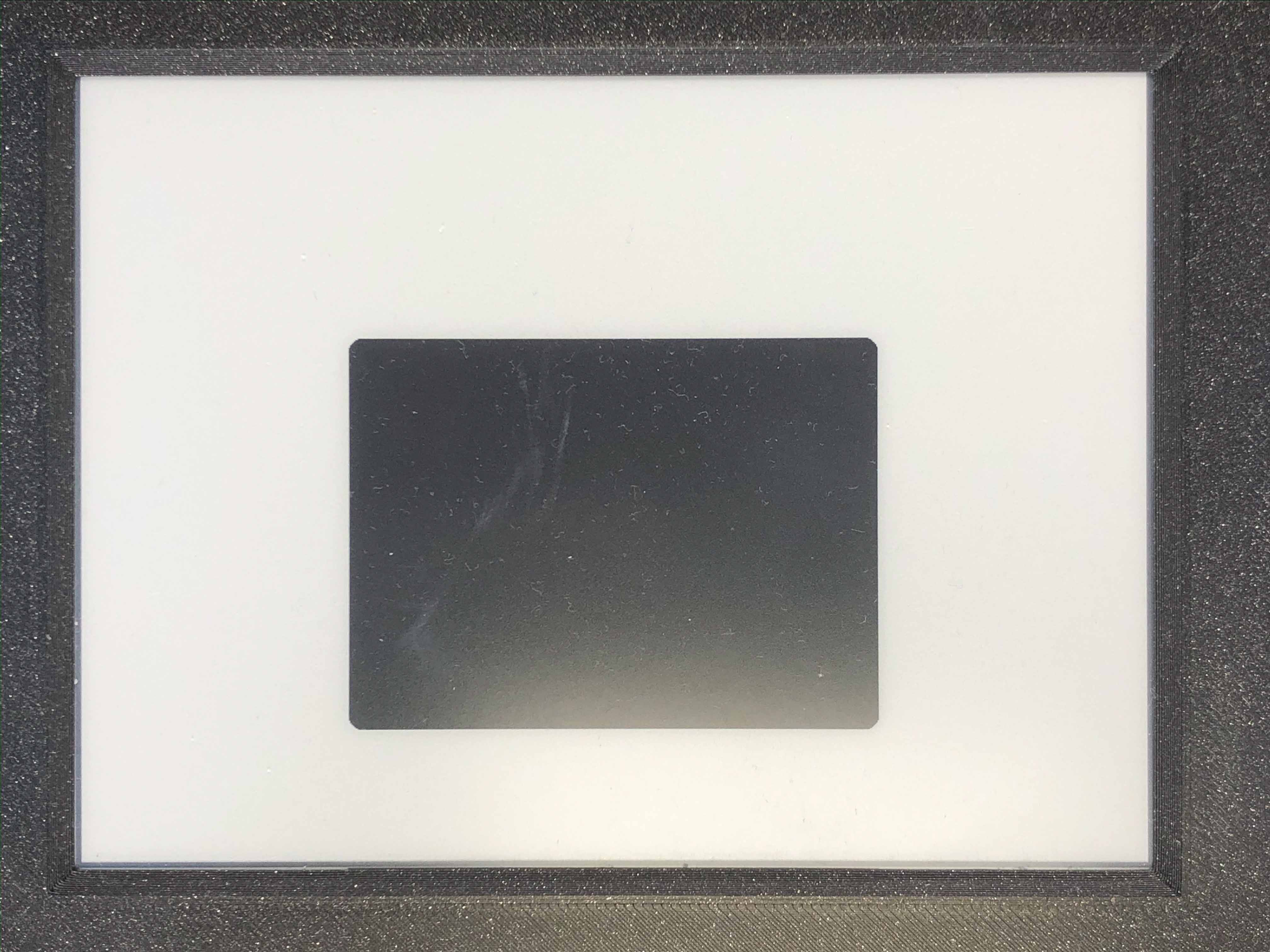### 3.2.2.30. Inkplate::drawBitmap();¶

• Method prototype (as seen in Adafruit_GFX.h):
```void drawBitmap(int16_t x, int16_t y, const uint8_t bitmap[],
int16_t w, int16_t h, uint16_t color);

void drawBitmap(int16_t x, int16_t y, const uint8_t bitmap[],
int16_t w, int16_t h, uint16_t color, uint16_t bg);

void drawBitmap(int16_t x, int16_t y, uint8_t *bitmap,
int16_t w, int16_t h, uint16_t color);

void drawBitmap(int16_t x, int16_t y, uint8_t *bitmap,
int16_t w, int16_t h, uint16_t color, uint16_t bg);
```
• Arguments and return value:
int16_t x - Bitmap x coordinate.
int16_t y - Bitmap y coordinate.
const uint8_t bitmap [] - Buffer storing the image information.
int16_t w - Bitmap width.
int16_t h - Bitmap height.
uint16_t color - Color to draw pixels marked with a 1.

int16_t x - Bitmap x coordinate.
int16_t y - Bitmap y coordinate.
const uint8_t bitmap [] - Buffer storing the image information.
int16_t w - Bitmap width.
int16_t h - Bitmap height.
uint16_t color - Color to draw pixels marked with a 1.
uint16_t bg - Color to draw pixels marked with a 0.

int16_t x - Bitmap x coordinate.
int16_t y - Bitmap y coordinate.
const uint8_t *bitmap - Buffer storing the image information.
int16_t w - Bitmap width.
int16_t h - Bitmap height.
uint16_t color - Color to draw pixels marked with a 1.

int16_t x - Bitmap x coordinate.
int16_t y - Bitmap y coordinate.
const uint8_t *bitmap - Buffer storing the image information.
int16_t w - Bitmap width.
int16_t h - Bitmap height.
uint16_t color - Color to draw pixels marked with a 1.
uint16_t bg - Color to draw pixels marked with a 0.

Returns nothing.

• Description:
Draws a monochrome bitmap to screen.
To get image data, use LCD image Converter.
• Example:
```display.drawBitmap(100, 250, logo, 576, 100, BLACK);
```
• Result:
Here is what the code above produces: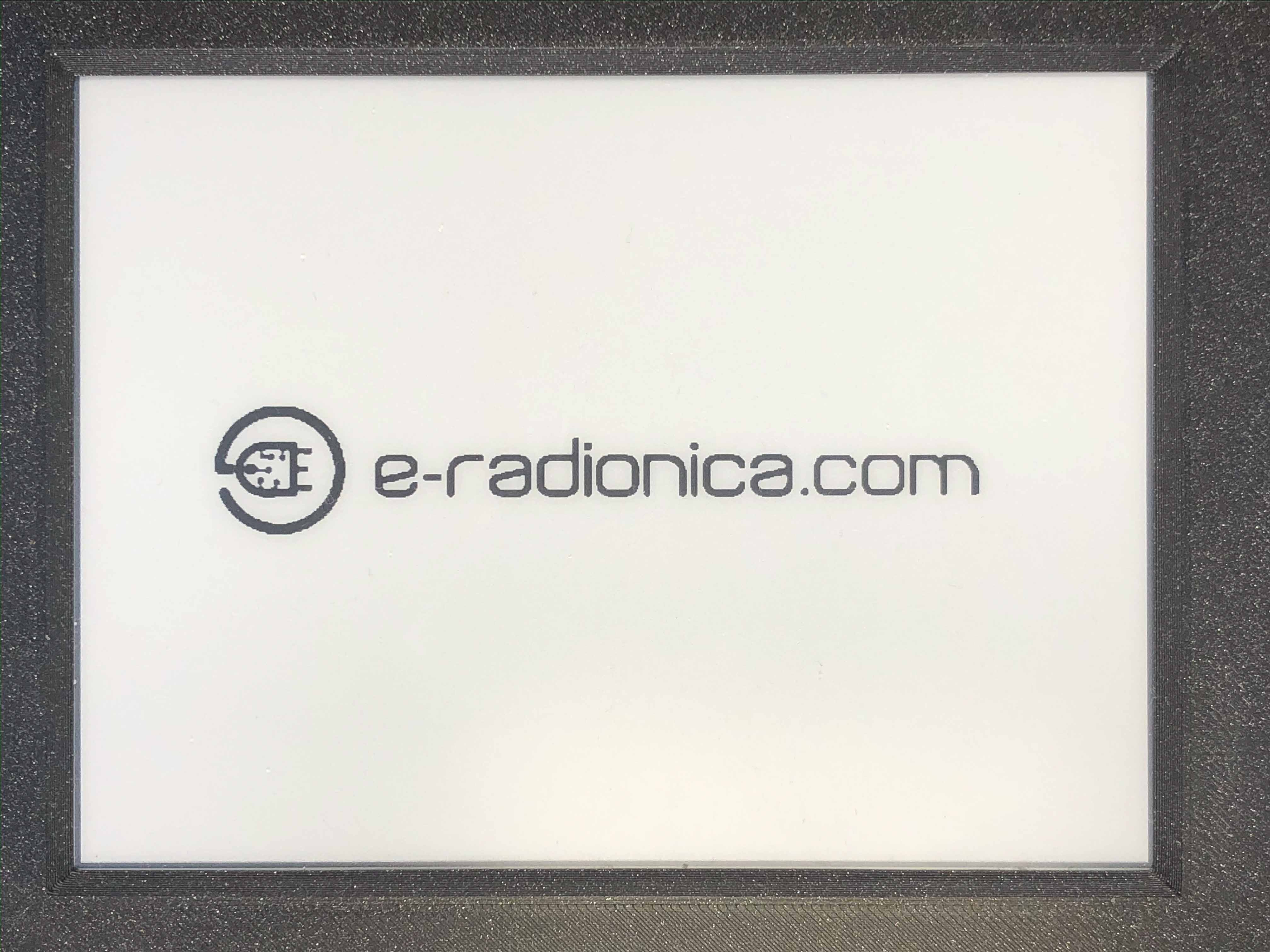### 3.2.2.31. Inkplate::drawBitmap3Bit();¶

• Method prototype (as seen in Image.h):
```void drawBitmap3Bit(int16_t _x, int16_t _y, const unsigned char *_p, int16_t _w, int16_t _h);
```
• Arguments and return value:
int16_t _x - x coordinate of image corner, [0, 799] in rotations 2, 4 and [0, 599] in 1, 3
int16_t _y - y coordinate of image corner, [0, 599] in rotations 2, 4 and [0, 799] in 1, 3
const unsigned char *_p - unsigned char buffer containing bitmap data
int16_t _w - image width
int16_t _h - image height

Returns nothing.

• Description:
Draws a bitmap image to screen.
Image data can be created using online tools or supplied Python script in some examples.
• Example:
```//Picture is a predefined image buffer (const uint8_t, see 2-Inkplate_basic_grayscale example)
display.drawBitmap3Bit(0, 0, picture, 800, 600);
```
• Result:
Here is what the code above produces: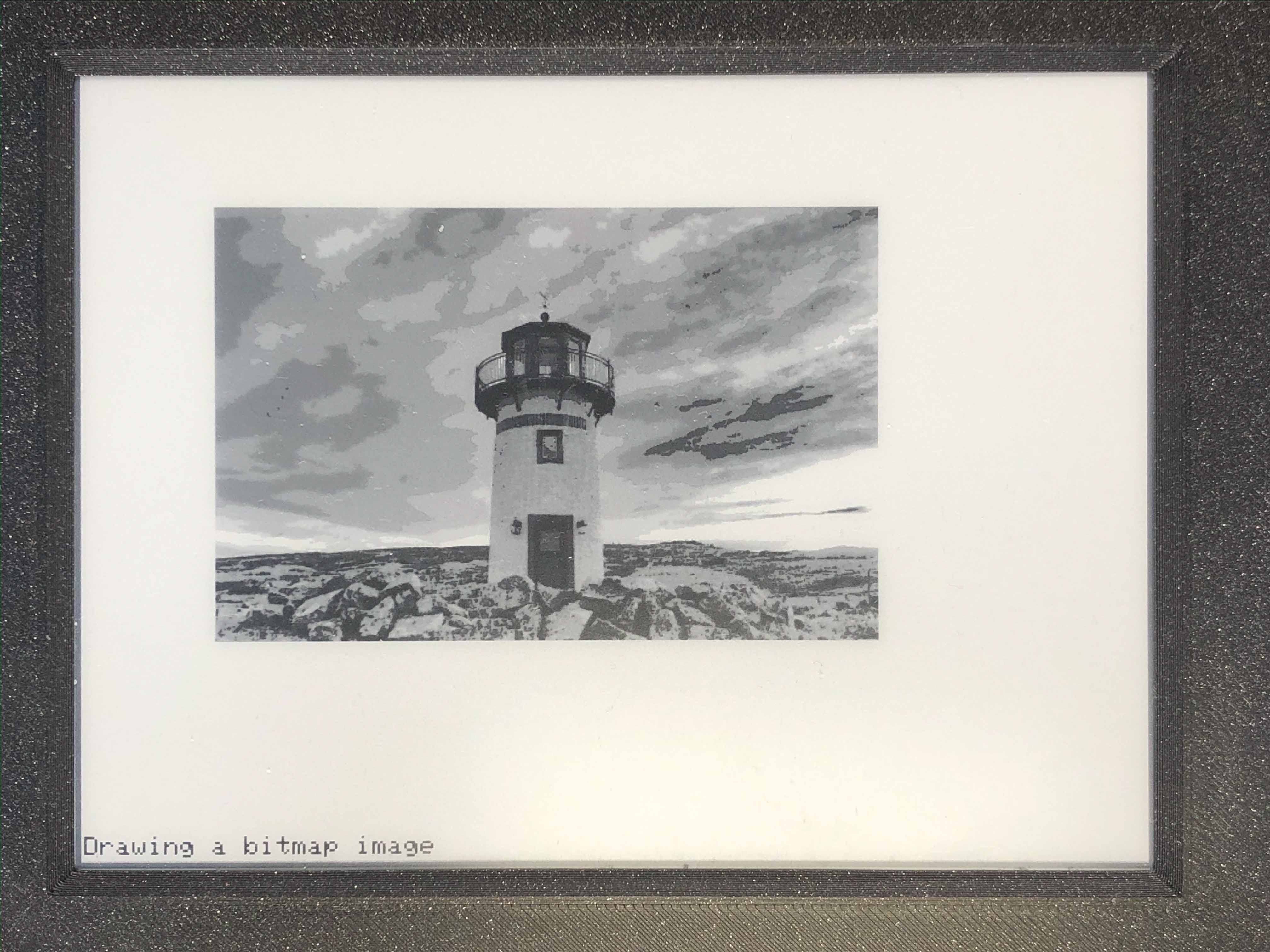### 3.2.2.32. Inkplate::drawChar();¶

• Method prototype (as seen in Adafruit_GFX.h):
```void drawChar(int16_t x, int16_t y, unsigned char c, uint16_t color,
uint16_t bg, uint8_t size);
void drawChar(int16_t x, int16_t y, unsigned char c, uint16_t color,
uint16_t bg, uint8_t size_x, uint8_t size_y);
```

### 3.2.2.33. Inkplate::getTextBounds();¶

• Method prototype (as seen in Adafruit_GFX.h):
```void getTextBounds(const char *string, int16_t x, int16_t y,
int16_t *x1, int16_t *y1, uint16_t *w, uint16_t *h);
void getTextBounds(const __FlashStringHelper *s, int16_t x, int16_t y,
int16_t *x1, int16_t *y1, uint16_t *w, uint16_t *h);
void getTextBounds(const String &str, int16_t x, int16_t y,
int16_t *x1, int16_t *y1, uint16_t *w, uint16_t *h);
```
• Arguments and return value:
const char *string - Text string from a char buffer (c style string - preferred).
int16_t x - Starting x coordinate.
int16_t y - Starting y coordinate.
int16_t *x1 - Pointer showing where to put end x coordinate.
int16_t *y1 - Pointer showing where to put end y coordinate.
uint16_t *w - Pointer showing where to put text width.
uint16_t *h -Pointer showing where to put text height.

const __FlashStringHelper *s - Text string from a flash string (preferred when string doesn’t have to be changed)
int16_t x - Starting x coordinate.
int16_t y - Starting y coordinate.
int16_t *x1 - Pointer showing where to put end x coordinate.
int16_t *y1 - Pointer showing where to put end y coordinate.
uint16_t *w - Pointer showing where to put text width.
uint16_t *h -Pointer showing where to put text height.

String &**string** - Text string from a String object reference (should be avoided).
int16_t x - Starting x coordinate.
int16_t y - Starting y coordinate.
int16_t *x1 - Pointer showing where to put end x coordinate.
int16_t *y1 - Pointer showing where to put end y coordinate.
uint16_t *w - Pointer showing where to put text width.
uint16_t *h -Pointer showing where to put text height.

Returns nothing.

• Description:
Puts data about text box end coordinates, width and height in variable pointers.
• Example:
```int16_t x, y;
uint16_t w, h;

display.getTextBounds("Some text", 0, 0, &x, &y, &w, &h);

// Now x, y, w and h were set to respected values
```

### 3.2.2.34. Inkplate::setTextSize();¶

• Method prototype (as seen in Adafruit_GFX.h):
```void setTextSize(uint8_t s);
void setTextSize(uint8_t sx, uint8_t sy);
```
• Arguments and return value:
uint8_t s - font scale

uint8_t sx - font x scale
uint8_t sy - font y scale

Returns nothing.

• Description:
Scales the font to some value.
• Example:
```display.setTextSize(4);
display.print("Welcome to Inkplate 6!");
```

### 3.2.2.35. Inkplate::setFont();¶

• Method prototype (as seen in Adafruit_GFX.h):
```void setFont(const GFXfont *f = NULL);
```
• Arguments and return value:
const GFXfont *f - font struct pointer, defaults to NULL meaning default font

Returns nothing.

• Description:
Used to change the text font.
Fonts can be found in the supplied Fonts folder or made using tools.
Example tool: font converter (select Library version -> gfx font)
• Example:
```//Font has to be included
display.setFont(&Not_Just_Groovy20pt7b);
display.println("Inkplate 6");
```
• Result:
Here is what the code above produces: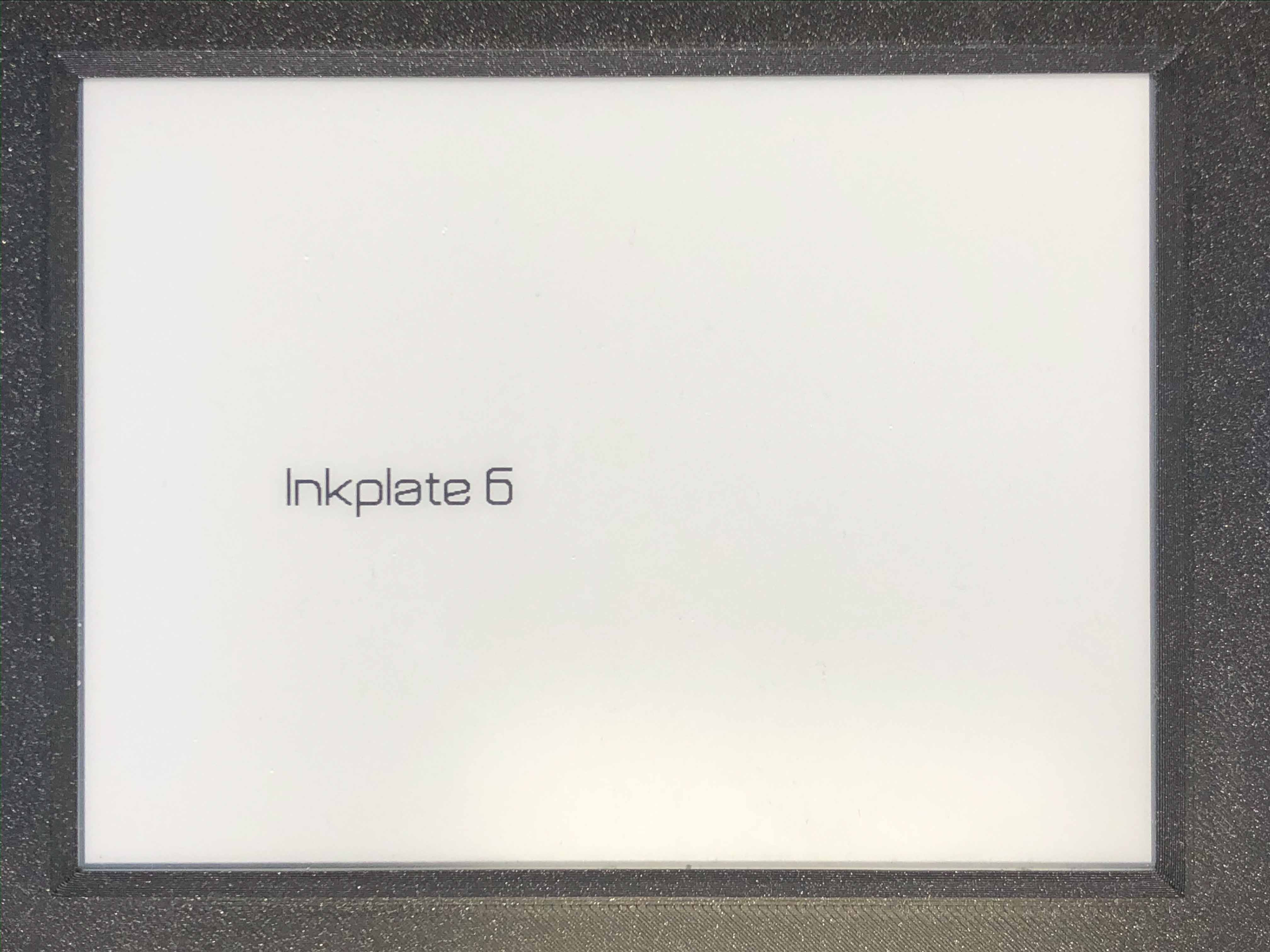### 3.2.2.36. Inkplate::setCursor();¶

• Method prototype (as seen in Adafruit_GFX.h):
```void setCursor(int16_t x, int16_t y);
```
• Arguments and return value:
int16_t x - Cursor x position.
int16_t y - Cursor y position.

Returns nothing.

• Description:
Sets the cursor text position.
• Example:
```display.setCursor(0, 550);
display.print("Some text");
```
• Result:
Here is what the code above produces: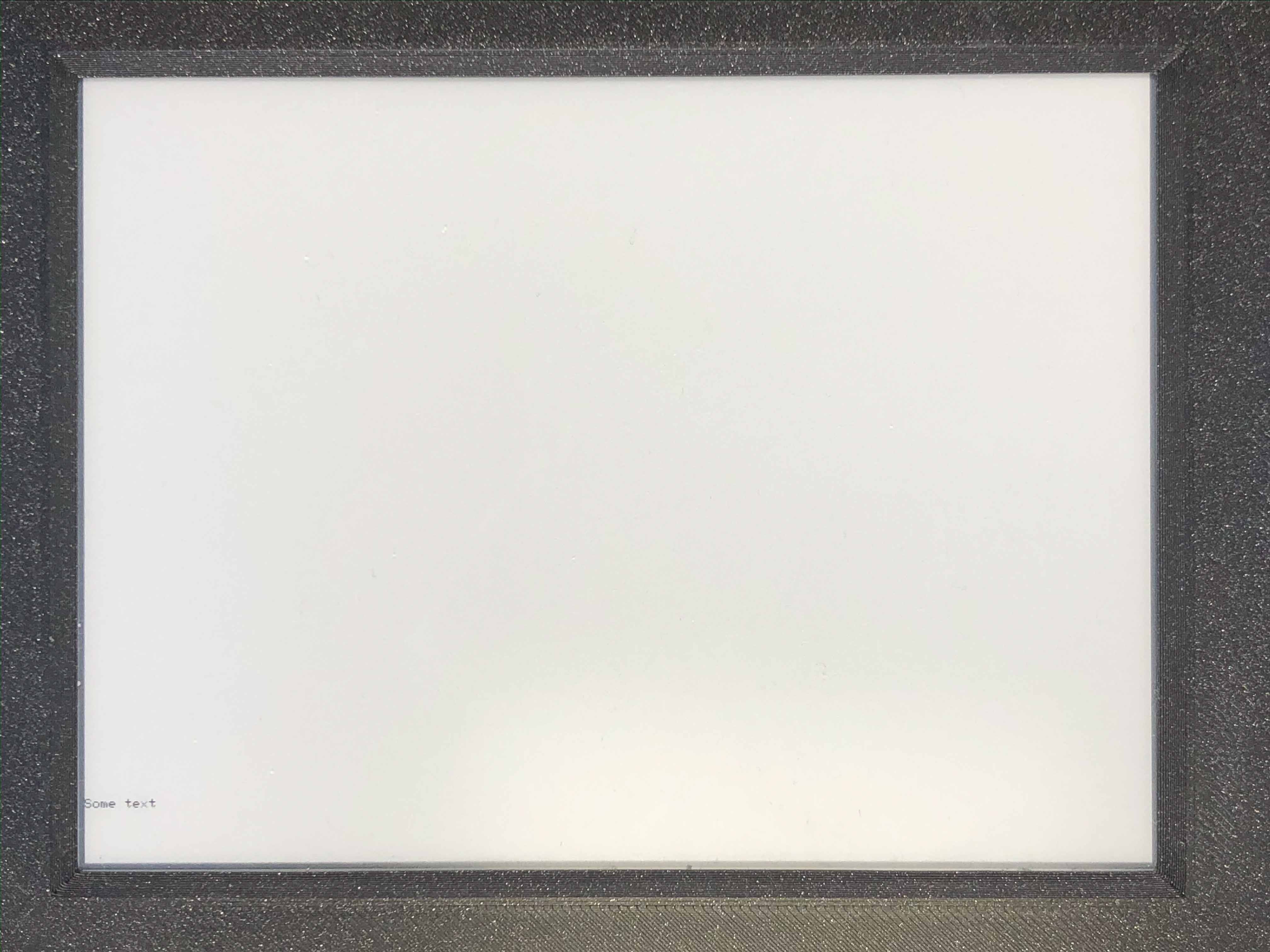### 3.2.2.37. Inkplate::print();¶

• Method prototype:
```void print(char *s);
void print(String &s);
```
• Arguments and return value:
char *s - C string to be printed.

String &**s** - String to be printed.

Returns nothing.

• Description:
Puts the text on screen.
• Example:
```display.print("Some text");
```
• Result:
Here is what the code above produces:### 3.2.2.38. Inkplate::println();¶

• Method prototype:
```void println(char *s);
void println(String &s);
```
• Arguments and return value:
char *s - C string to be printed.

String &**s** - String to be printed.

Returns nothing.

• Description:
Essentially the same as print but adds a new line to end.
• Example:
```display.println("Some text");
```

### 3.2.2.39. Inkplate::setTextWrap();¶

• Method prototype (as seen in Adafruit_GFX.h):
```void setTextWrap(boolean w);
```
• Arguments and return value:
boolean w - to wrap or not to wrap text.

Returns nothing.

• Description:
Wrap text thats gone of the edge.
• Example:
```//Disables text wrapping
display.setTextWrap(false);
```

### 3.2.2.40. Inkplate::width();¶

• Method prototype (as seen in Graphics.h):
```int16_t width(void);
```
• Arguments and return value:
No arguments.
• Description:
Returns screen width.
• Example:
```display.width();
```

### 3.2.2.41. Inkplate::height();¶

• Method prototype (as seen in Graphics.h):
```int16_t height(void);
```
• Arguments and return value:
No arguments.

Returns nothing.

• Description:
Returns screen height.
• Example:
```display.height();
```

### 3.2.2.42. Inkplate::getRotation();¶

• Method prototype (as seen in Graphics.h):
```int16_t getRotation(void);
```
• Arguments and return value:
No arguments.

Returns nothing.

• Description:
Returns screen rotation, in range [0,3], 2 is default.
• Example:
```if(display.getRotation() == 4)
Serial.println("I'm upside down!");
```

### 3.2.2.43. Inkplate::getCursorX();¶

• Method prototype (as seen in Adafruit_GFX.h):
```int16_t getCursorX(void);
```
• Arguments and return value:
No arguments.

Returns nothing.

• Description:
Returns text cursor x coordinate.
• Example:
```if(display.getCursorX() > 400)
Serial.println("Were in the second half of the screen!");
```

### 3.2.2.44. Inkplate::getCursorY();¶

• Method prototype (as seen in Adafruit_GFX.h):
```int16_t getCursorY(void);
```
• Arguments and return value:
No arguments.

Returns nothing.

• Description:
Returns text cursor y coordinate.
• Example:
```if(display.getCursorY() > 300)
Serial.println("Were in the bottom half of the screen!");
```

## 3.2.3. MCP Functions¶

MCP23017 Expander PORTB pins from GPB1-GPB7 can be used.
DO NOT USE GPA0-GPA7 and GPB0. In code those are pins from 0-8.
Using those, you might permanently damage the screen.
You should only use pins from 9-15.
Port A is used for epaper panel and TPS65186 PMIC. GPB0 is used for ESP32 GPIO0 so you can’t use it either.
GPB1 is used for enabling battery reading (if Batt solder bridge is bridged between second and third pad)
MCP is started inside Inkplate.begin() function so you need only to call that and everything is set for MCP.
```Inkplate display(INKPLATE_1BIT);//or INKPLATE_3BIT
display.begin();
```

### 3.2.3.1. Inkplate::pinModeMCP();¶

• Method prototype (as seen in Mcp.h):
```void pinModeMCP(uint8_t _pin, uint8_t _mode);
```
• Arguments and return value:
uint8_t _pin - pin number.
uint8_t _mode - mode to be set (INPUT, OUTPUT or INPUT_PULLUP).
Returns nothing.
• Description:
Sets internal pin mode.
• Example:
```display.pinModeMCP(LED_PIN, OUTPUT);
```

### 3.2.3.2. Inkplate::digitalWriteMCP();¶

• Method prototype (as seen in Mcp.h):
```void digitalWriteMCP(uint8_t _pin, uint8_t _state);
```
• Arguments and return value:
uint8_t _pin - pin number.
uint8_t _state - pin state (HIGH or LOW).
Returns nothing.
• Description:
Sets internal output pin state.
• Example:
```display.digitalWriteMCP(LED_PIN, HIGH);
```

• Method prototype (as seen in Mcp.h):
```uint8_t digitalReadMCP(uint8_t _pin);
```
• Arguments and return value:
uint8_t _pin - pin number.
Returns HIGH or LOW value (1 or 0).
• Description:
• Example:
```display.digitalReadMCP(LED_PIN);
```

### 3.2.3.4. Inkplate::setIntOutput();¶

• Method prototype (as seen in Mcp.h):
```void setIntOutput(uint8_t intPort, uint8_t mirroring, uint8_t openDrain, uint8_t polarity);
```
• Arguments and return value:
uint8_t intPort - intPort portA or portB.
uint8_t mirroring - mirroring set 1 to make ports mirror each other so that any interrupt will cause both to go HIGH.
uint8_t openDrain - openDrain set 1 to set interupt port as open drain, this will override port polarity.
uint8_t polarity - sets port interrupt polarity, 1 active high, 0 active low.
Returns nothing.
• Description:
Sets port interrupt state
• Example:
```display.setIntOutput(1, false, false, HIGH);// 1 means portB, 0 portA
```

### 3.2.3.5. Inkplate::setIntPin();¶

• Method prototype (as seen in Mcp.h):
```void setIntPin(uint8_t _pin, uint8_t _mode);
```
• Arguments and return value:
uint8_t _pin - pin number.
uint8_t mode - interurpt mode (CHANGE, FALLING, RISING)
Returns nothing.
• Description:
Sets pin interrupt state
• Example:
```display.setIntPin(touchPadPin, RISING);
```

### 3.2.3.6. Inkplate::removeIntPin();¶

• Method prototype (as seen in Mcp.h):
```void removeIntPin(uint8_t _pin);
```
• Arguments and return value:
uint8_t _pin - pin number.
Returns nothing.
• Description:
Removes interrupt from pin
• Example:
```display.removeIntPin(touchPadPin);
```

### 3.2.3.7. Inkplate::getINT();¶

• Method prototype (as seen in Mcp.h):
```uint16_t getINT();
```
• Arguments and return value:
No argument.
Returns interupt registers state.
• Description:
Returns interrupt registers state for portA and portB.
Every bit represents interrupt pin, MSB is PORTB PIN7, LSB is PORTA PIN1.
• Example:
```display.getINT();
```

### 3.2.3.8. Inkplate::getINTstate();¶

• Method prototype (as seen in Mcp.h):
```uint16_t getINTstate();
```
• Arguments and return value:
No argument.
Returns interupt registers state at the time interrupt occured.
• Description:
Returns interrupt registers state for portA and portB.
Every bit represents interrupt pin, MSB is PORTB PIN7, LSB is PORTA PIN1.
• Example:
```display.getINTstate();
```

### 3.2.3.9. Inkplate::setPorts();¶

• Method prototype (as seen in Mcp.h):
```void setPorts(uint16_t _d);
```
• Arguments and return value:
uint16_t _d - value to be writen to port A and port B registers.
Returns nothing.
• Description:
sets internal state of PORTA and PORTB registers.
MSB byte is for PORTB, LSB byte for PORTA
• Example:
```uint16_t data = 0xFFFF;//to make all bits ones
display.setPorts(data);
```

### 3.2.3.10. Inkplate::getPorts();¶

• Method prototype (as seen in Mcp.h):
```uint16_t getPorts();
```
• Arguments and return value:
No arguments.
Returns register state of PORTA and PORTB.
• Description:
returns internal state of PORTA and PORTB registers.
MSB byte is for PORTB, LSB is for PORTA.
• Example:
```display.getPorts();
```

## 3.2.4. NetworkClient Functions¶

### 3.2.4.1. WiFi connectivity¶

For some functionalities of the Inkplate to work you must be connected to WiFi.
```#include "Inkplate.h"
#include <WiFi.h>
#include <HTTPClient.h>

const char ssid = "Wifi_name"

...

//In setup
while(!display.joinAP(ssid, pass))
{
Serial.println("Connecting to wifi");
}

//after you can check if connection active
if((display.isConnected))
{
HTTPClient http;

http.begin("http://example.com/index.html");

int httpCode = http.GET();

if(httpCode > 0)
{
if(httpCode == HTTP_CODE_OK)
{

...
}
}
}
```

### 3.2.4.2. Inkplate::joinAP();¶

• Method prototype (as seen in NetworkClient.h):
```bool joinAP(const char *ssid, const char *pass);
```
• Arguments and return value:
const char *ssid - name of the wifi network.
const xhar *pass - network password.
Returns 1 if successfuly connected, 0 if not.
• Description:
Sets and connects inkplate to on wifi network.
• Example:
```//In setup
while(!display.joinAP(ssid, pass))
{
Serial.println("Connecting to wifi");
}
```

### 3.2.4.3. Inkplate::disconnect();¶

• Method prototype (as seen in NetworkClient.h):
```void disconnect();
```
• Arguments and return value:
No arguments.
Returns nothing.
• Description:
Disconnects Inkplate from wifi network (shuts network).
• Example:
```display.disconnect();
```

### 3.2.4.4. Inkplate::isConnected();¶

• Method prototype (as seen in NetworkClient.h):
```bool isConnected();
```
• Arguments and return value:
No arguments.
Returns 1 if connected to wifi, 0 if not.
• Description:
Checks if inkplate is connected to wifi.
• Example:
```Serial.println(display.isConnected());
```

• Method prototype (as seen in NetworkClient.h):
```uint8_t *downloadFile(const char *url, int32_t *defaultLen);
```
• Arguments and return value:
const char *url - link to file.
int32_t *defaultLen - expected lenght (only matters if real length cant be checked).
Returns file as byte buffer, NULL if failed to get file.
WiFiClient *url - link to file
int32_t *len - expected lenght (only matters if real length cant be checked).
Returns file as byte buffer, NULL if failed to get file.
• Description:
```char url = "https//:www.somepic.com/pic.jpg"